# Using flashlight

#### 2021-01-10

library(ggplot2)
library(flashlight)      # model interpretation
library(MetricsWeighted) # metrics
library(dplyr)           # data prep
library(moderndive)      # data
library(rpart)           # used if XGBoost not available
library(ranger)          # random forest

has_xgb <- requireNamespace("xgboost", quietly = TRUE)
if (!has_xgb) {
message("Since XGBoost is not available, will use rpart.")
}

## Introduction

In contrast to classical statistical modelling techniques like linear regression, modern machine learning approaches tend to provide black box results. In areas like visual computing or natural language processing, this is not an issue since their focus is usually on predicting things. Either the predictions are sufficiently useful in practice or the model won’t be used. However, in areas where the purpose of a statistical model is also to explain or validate underlying theories (e.g. in medicine, economics, and biology), black box models are of little use.

Thus, there is need to shed light into these black boxes resp. to explain machine learning models as good as possible. An excellent reference is the online book (Molnar 2019). Of special interest are model agnostic approaches that work for any kind of modelling technique, e.g. a linear regression, a neural net or a tree-based method. The only requirement is the availability of a prediction function, i.e. a function that takes a data set and returns predictions.

This is the purpose of the R package flashlight, which is inspired by the beautiful DALEX package, see (https://CRAN.R-project.org/package=DALEX).

The main props of flashlight:

1. It is simple, yet flexible.

2. It offers model agnostic tools like model performance, variable importance, global surrogate models, ICE profiles, partial dependence, further effects plots, scatter plots, interaction strength, and variable contribution breakdown for single observations.

3. It allows to assess multiple models in parallel without any redundancy in the code.

4. It supports “group by” operations.

5. All methods are able to utilize case weights.

Currently, models with numeric or binary response are supported.

We will now give a brief introduction to machine learning explanations and then illustrate them with the flashlight package.

## Background

Important model agnostic machine learning explanations include the following aspects, amongst many others.

### Model performance

How precise are models if applied to unseen data? This aspect is of key interest of basically any supervised machine learning model and helps to identify the best models or, if applied to subgroups, identify problematic segments with low performance.

### Variable importance

Which variables are particularly relevant for the model? This aspect is helpful in different ways. Firstly, it might help to simplify the full modelling process by eliminating difficult to assess input variables with low explanatory power. Secondly, its pure information. Thirdly, it might help to identify problems in data structure: if one variable is extremely relevant and all others are not, then there might be some sort of information leakage from the response. Finally, variable importance considerations are very relevant for quality assurance as well.

Different modelling techniques offer different ways of variable importance. In linear models, we consider for example F-test statistics or p values, in tree-based methods the number of splits or average split gains etc. A model agnostic way to assess this is called permutation importance: For each input variable $$X$$, its values are randomly shuffled and the drop in performance with respect to a scoring function is calculated. The more important a variable, the larger the drop. If a variable can be shuffled without any impact on model precision, it is completely irrelevant. The method is described in (Fisher, Rudin, and Dominici 2018). Multiple permutations lead to more stable estimates of variable importance and allow the calculation of standard errors.

### Effects of input variables

In linear regression, the fitted model consists of an affine linear function in the inputs. Its coefficients immediately tell us how the response is expected to change if the value of one single input variable $$X$$ is systematically being adapted. How to describe such effects of a variable $$X$$ for more complex models that include non-linearities and high-order interactions?

One approach is to study Individual Conditional Expectation (ICE) profiles of selected observations: They show how predictions of observation $$i$$ react when the input variable $$X$$ is systematically being changed, see (Goldstein et al. 2015). The more different the profiles are in shape or slope, the stronger are the interaction effects. For a linear regression without interactions, all such profiles would be parallel. Centered ICE profiles (“c-ICE”) help to detect interactions even better.

If many ICE profiles are averaged, we get partial dependence profiles which can be viewed as the average effect of variable $$X$$, pooled over all interactions. Partial dependence plots where introduced in Friedman’s seminal 2001 article on gradient boosting (Friedman 2001). Partial dependence profiles (as well as ICE profiles) are not limited to one single variable $$X$$. Also multiple variables can be systematically varied on a grid in order to study the multivariate impact on the typical response.

Studying ICE and partial dependence profiles means investigating the effect of $$X$$ while holding all other predictors fixed. The more unnatural this Ceteris Paribus assumption is, the less reasonable are the results of ICE and partial dependence. Accumulated local effects or ALE profiles (Apley and Zhu 2016) try to overcome this weakness of ICE and partial dependence considerations.

ALE profiles at positions $$x_i$$ are calculated as follows:

1. First, left derivatives $$\Delta_i$$ are estimated for all $$i$$: Calculate slope of partial dependence line from $$x_i-\varepsilon$$ to $$x$$ based on data points in $$[x_i-\varepsilon, x_i]$$.

2. The uncalibrated ALE value at $$x_i$$ is the (integrated/accumulated) partial sum $$\sum_{j \le i} \Delta_j$$.

3. In the calibration step, the effects are shifted to the level of the response variable or its predictions.

An alternative to partial dependence and ALE profiles is to look at combined effects of $$X$$ including the effects from all other predictors. Such effects are estimated by averaging the predictions within values of the predictor $$X$$ of interest. If such prediction profile differs considerably from the observed average response, this might be a sign of model underfit. This visualization is sometimes called “marginal plot” or “M plot”, see (Apley and Zhu 2016). Residual profiles immediately show such misfits as well. In classical statistical modelling, this sort of plots are called “fitted versus covariable” plots or “residual versus covariable” plots.

If SHAP values have been calculated, plotting their average contributions of $$X$$ against $$X$$ is an elegant way to visualize the effect of $$X$$, see Subsection “Variable contribution breakdown for single observations”.

Besides looking at average profiles, it is often also revealing to consider quartiles or to visualize partial dependence, response and prediction profiles in the same plot.

Instead of studying profiles, it might be revealing to look at scatter plots of responses, predictions, residuals, or SHAP values versus $$X$$.

### Interaction strength

Besides measuring overall variable importance, an interesting aspect is to measure the strength of non-additivity associated with each covariable (i.e. the overall interaction strength) and/or between pairs of covariables. A model-agnostic way to assess such measures is based on partial dependence curves, see (Friedman and Popescu 2008). A computationally less demanding alternative to their H-statistic of measuring total interaction strength per covariable can be extracted from centered ICE: How much of their variability is unexplained by the main effect?

### Variable contribution breakdown for single observations

Instead of studying global variable importance and effects, there are different techniques to entangle how a single prediction can be explained: LIME, LIVE, SHAP and breakdown, see (Molnar 2019) and (Gosiewska and Biecek 2019) for an overview. The flashlight package currently supports the breakdown method as well as approximate SHAP. These two methods are based on additive decomposition of a prediction.

The breakdown algorithm works as follows: First, the visit order $$(x_1, ..., x_m)$$ of variables is specified. Then, in the query data, the column $$x_1$$ is set to the constant value of $$x_1$$ of the observation to be explained. The change in the (weighted) average predicted value on the query data measures the contribution of $$x_1$$ on the prediction. This procedure is iterated over all $$x_i$$ until eventually, all rows in the query data are identical to the observation to be explained.

A complication with this approach is that the visit order is relevant, at least for non-additive models. Ideally, the algorithm could be repeated for all $$m!$$ possible visit orders and its results averaged per variable. This is what SHAP does, see e.g. (Gosiewska and Biecek 2019) for an explanation. Unfortunately, there is no efficient way to do this in a model agnostic way. Thus we offer two approximations. The first one is the short-cut described in (Gosiewska and Biecek 2019) and implemented in the ibreakdown package (https://CRAN.R-project.org/package=iBreakDown): There, the variables $$x_i$$ are sorted by the size of their contribution in the same way as the breakdown algorithm but without iteration, i.e. starting from the original query data for each variable $$x_i$$. We call this approach “breakdown”. The second, computationally more intensive way to approximate SHAP values is based on running the breakdown algorithm on a small number (e.g. 12) random permutations and then averaging the results. We call this approach (approximate) “SHAP”.

### Global surrogate models

As suggested in (Molnar 2019), one way to explain a complex model is to fit an easy to interprete model like a decision tree to the predictions of the complex model and then study this simple surrogate model. The quality of its approximation can be measured by the R-squared of the surrogate model.

### flashlight

The flashlight package offers these tools in a very simple way.

## Installation of flashlight

From CRAN:

install.packages("flashlight")

library(devtools)
install_github("mayer79/flashlight")

## Teaser

Let’s start with an iris example. For simplicity, we do not split the data into training and testing/validation sets.

# Fit model
fit <- lm(Sepal.Length ~ ., data = iris)

# Make flashlight
fl <- flashlight(model = fit, data = iris, y = "Sepal.Length", label = "ols",
metrics = list(rmse = rmse, R-squared = r_squared))

# Performance: rmse and R-squared
plot(light_performance(fl), fill = "darkred")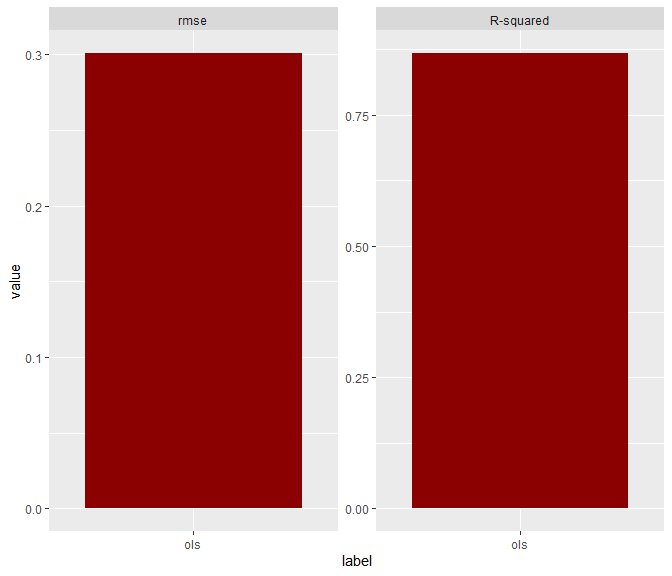plot(light_performance(fl, by = "Species"), fill = "darkred")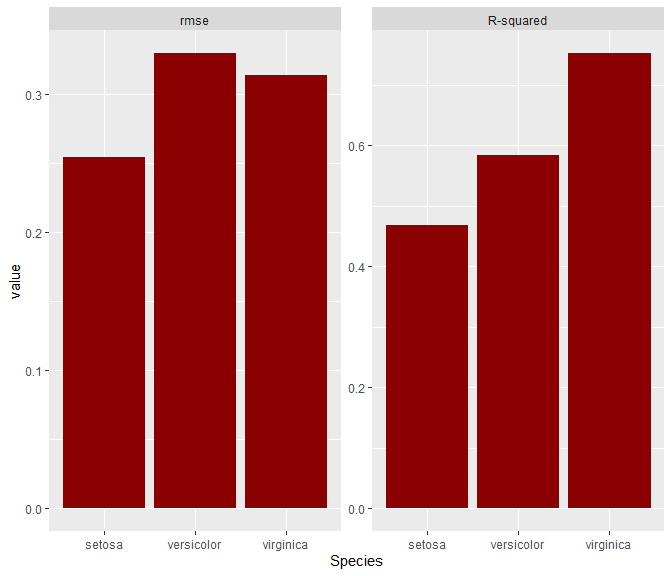# Variable importance by increase in rmse
imp <- light_importance(fl, m_repetitions = 4)
plot(imp, fill = "darkred")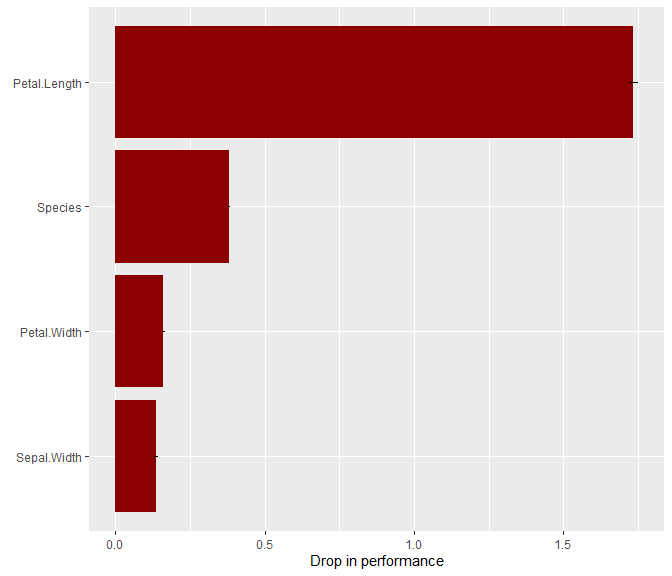plot(light_importance(fl, by = "Species"))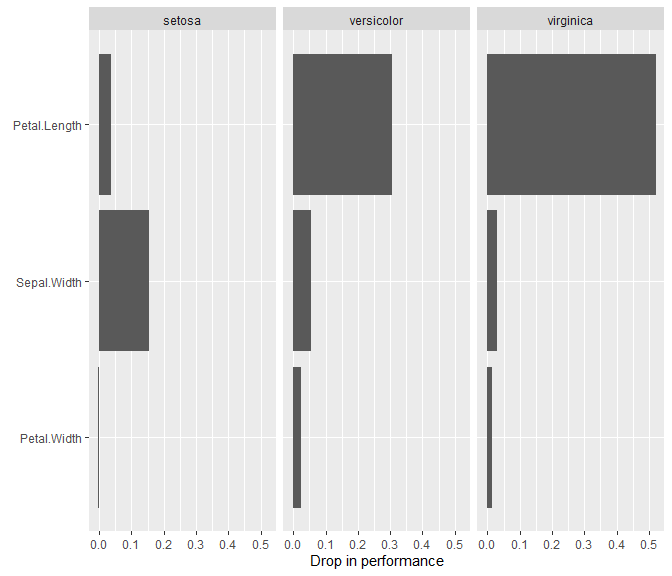most_important(imp, 2)
#>  "Petal.Length" "Species"

# ICE profiles for Petal.Width
plot(light_ice(fl, v = "Petal.Width"))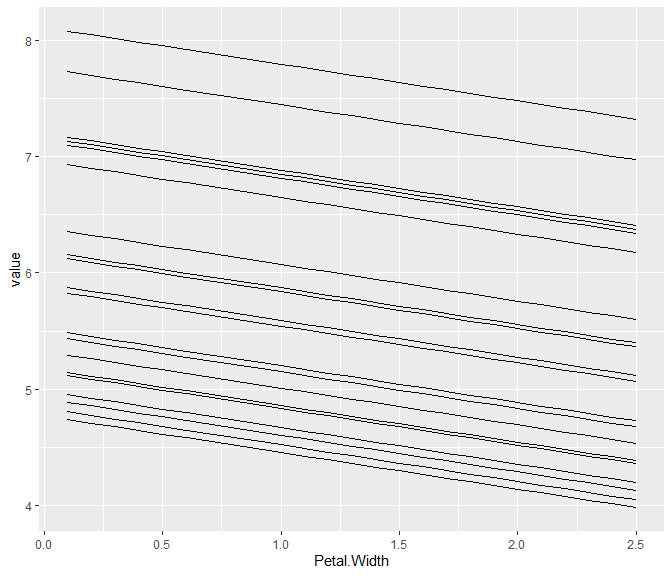plot(light_ice(fl, v = "Petal.Width", center = "first"))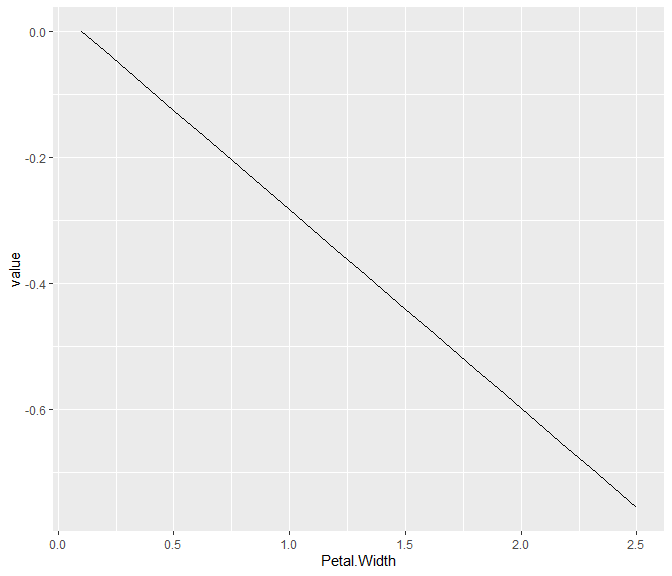plot(light_ice(fl, v = "Petal.Width", by = "Species"))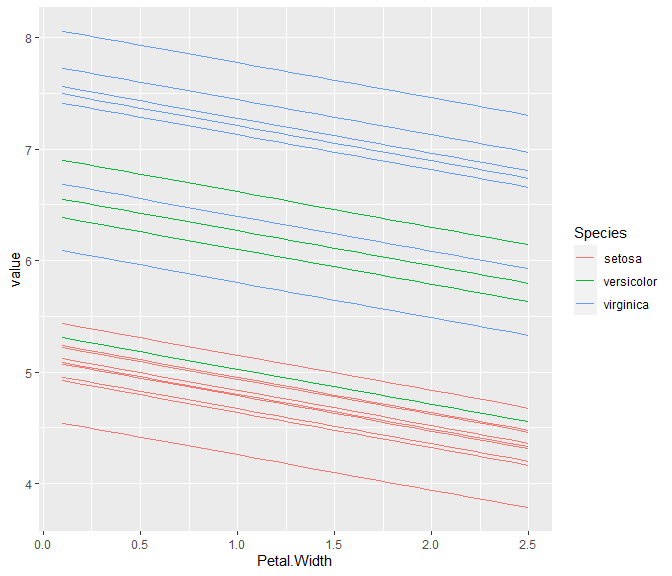# Partial dependence profiles for Petal.Width
plot(light_profile(fl, v = "Petal.Width"))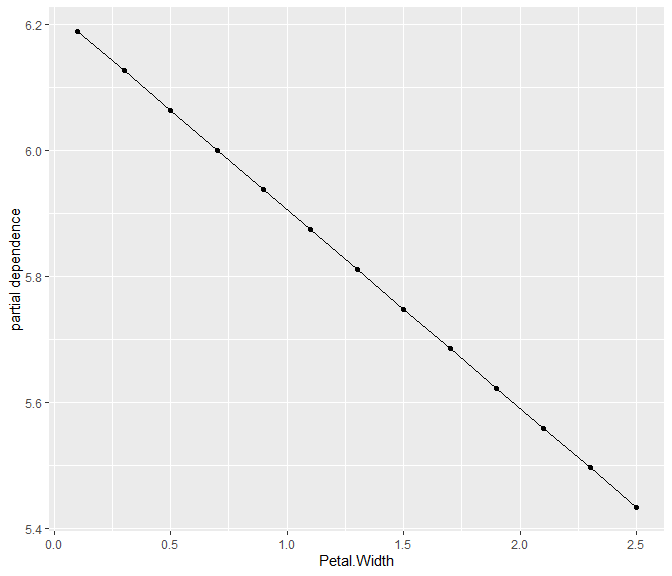plot(light_profile(fl, v = "Petal.Width", by = "Species"))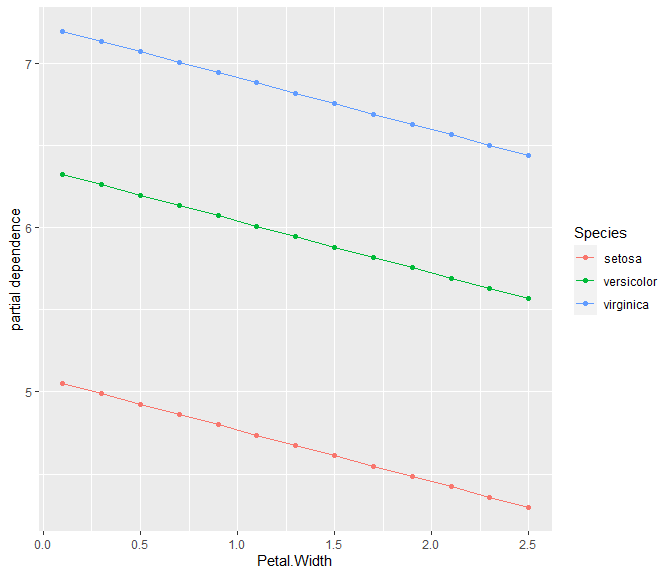# Accumulated local effects (ALE) profiles for Petal.Width
plot(light_profile(fl, v = "Petal.Width", type = "ale"))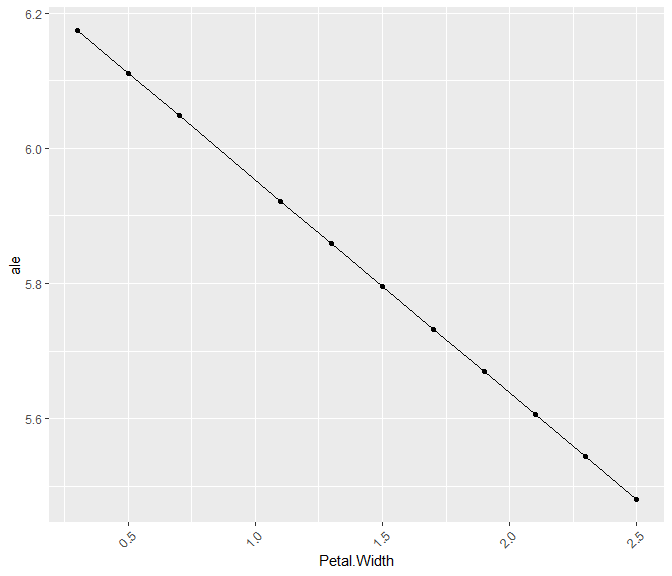plot(light_profile(fl, v = "Petal.Width", by = "Species", type = "ale"))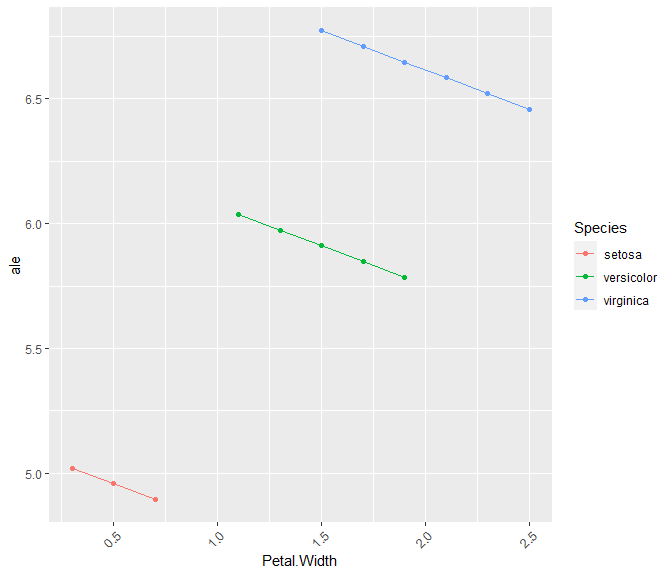# Prediction, response and residual profiles
plot(light_profile(fl, v = "Petal.Width", type = "response", stats = "quartiles"))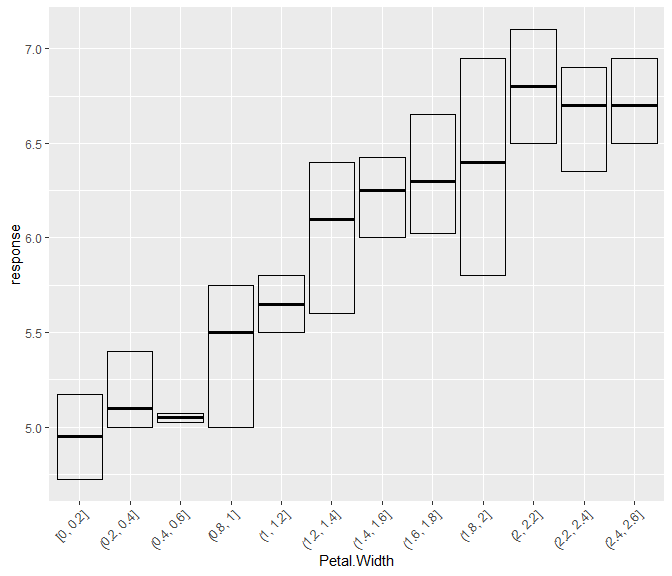plot(light_profile(fl, v = "Petal.Width", type = "predicted"))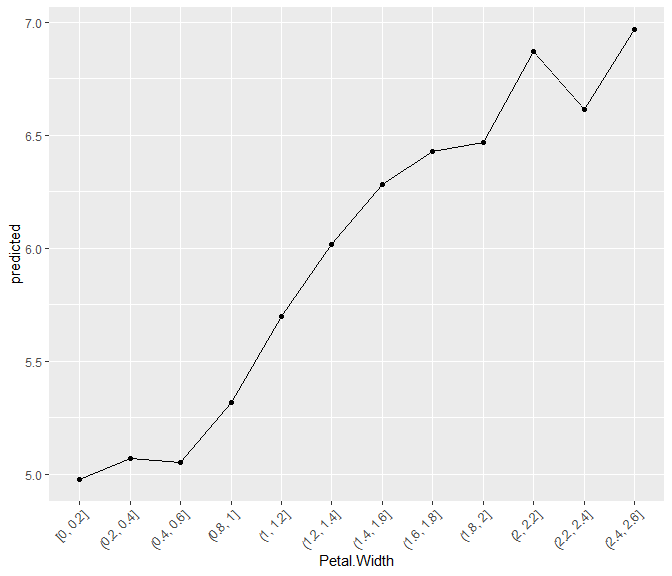plot(light_profile(fl, v = "Petal.Width", type = "residual", stats = "quartiles"))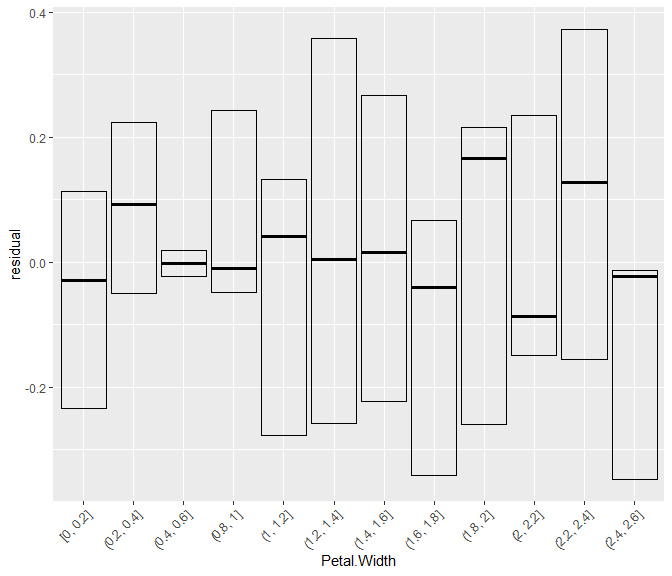# Response profiles, prediction profiles, partial dependence, and ALE profiles in one
plot(light_effects(fl, v = "Petal.Width"), use = "all")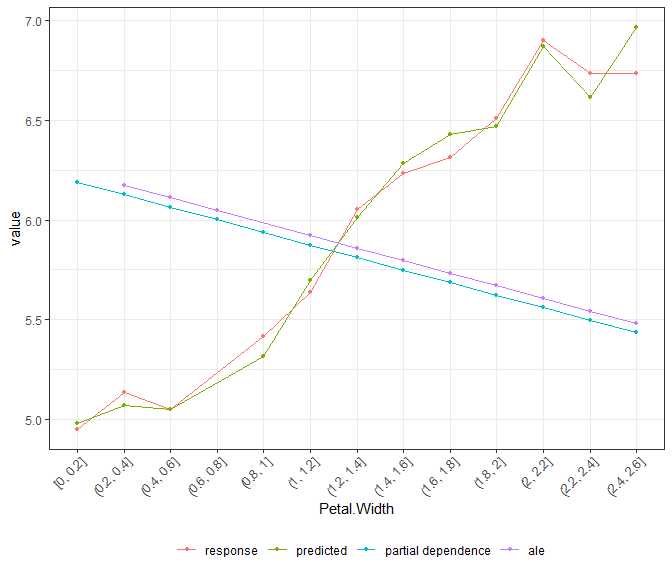# Scatter plots
plot(light_scatter(fl, v = "Petal.Width", type = "predicted"))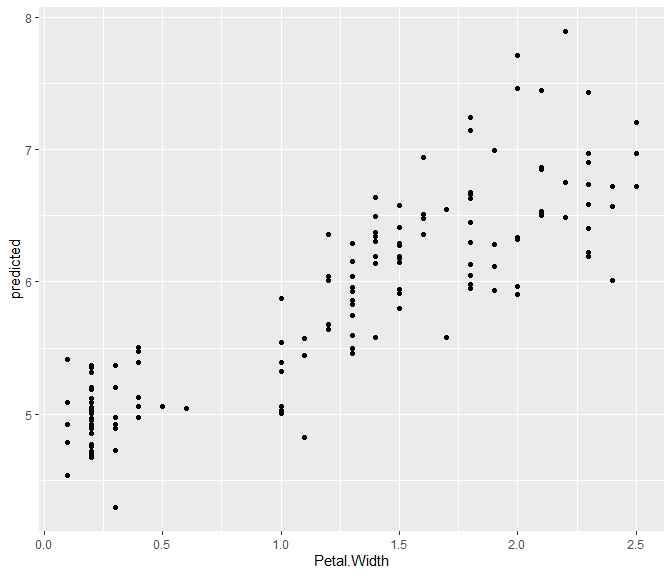# Variable contribution breakdown for single observation
plot(light_breakdown(fl, new_obs = iris[2, ]))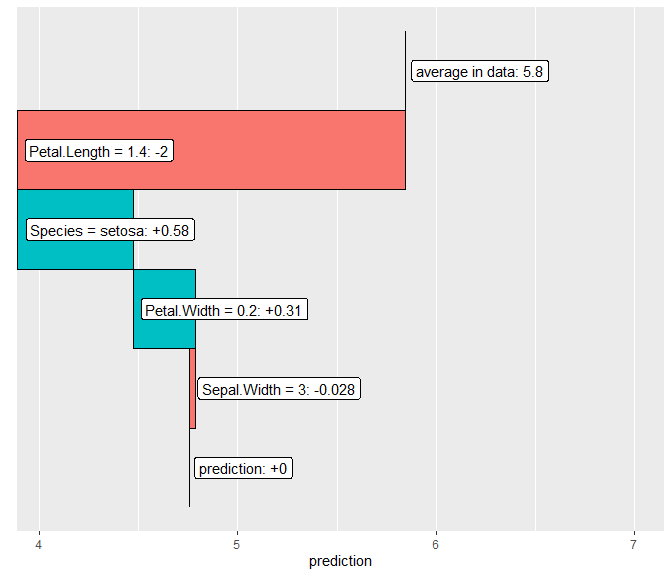# Global surrogate
plot(light_global_surrogate(fl))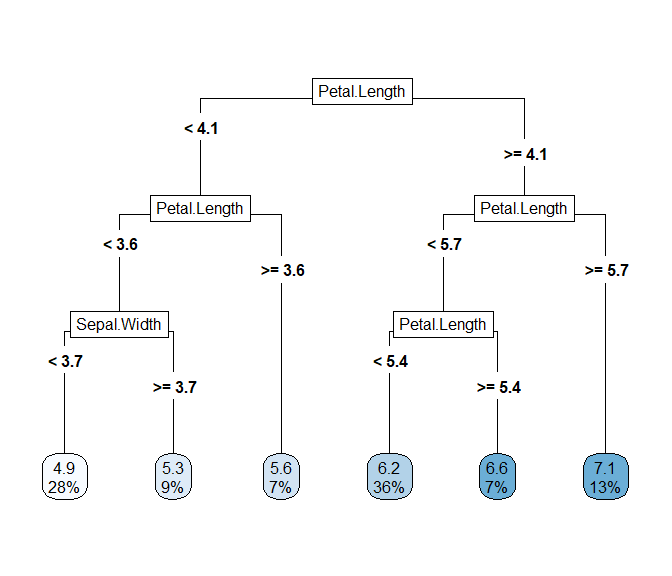## flashlights and multiflashlights

The process of using the flashlight package is as follows:

1. Define a flashlight object for each model. This is basically a list with optional components relevant for model interpretation:

• model: The fitted model object as the one returned by lm.

• data: A data set used to evaluate model agnostic tools, e.g. the validation data.

• y: The name of the variable in data representing the model response.

• predict_function: A function taking model and data and returning numeric predictions.

• linkinv: Inverse link function used to retransform the values returned by predict_function. Defaults to the identity function function(z) z.

• w: The name of the variable in data representing the case weights.

• by: A character vector of names of grouping variables in data. These will be used to stratify all results.

• metrics: A named list of metrics. These functions need to be available in the workspace and require arguments actual, predicted, w (case weights) as well as a placeholder … for further arguments. All metrics available in R package MetricsWeighted are suitable.

• label: The name of the model as shown in plots. This is the only required input when building the flashlight.

2. Calculate relevant information by calling the key functions:

• light_performance: Calculates performance measures regarding different metrics, possibly within subgroups and weighted by case weights.

• light_importance: Calculates variable importance (worsening in performance by random shuffling) for each or a subset of variables. Possibly within subgroups and using case weights. The most important variable names can be extracted by the function most_important on the result of light_importance.

• light_ice: Calculates ICE profiles across a couple of observations, possibly within groups.

• light_scatter: Calculates values (e.g. predictions) to be plotted against a variable of interest.

• light_profile: Calculates partial dependence profiles across a covariable, possibly within groups. Generated by calling light_ice and aggregating the results. The function is flexible: it can also be used to generate ALE, response, residual, prediction, or SHAP profiles and calculate (weighted) quartiles instead of (weighted) means.

• light_effects: Combines partial dependence, response and prediction profiles. ALE profiles can be added as well.

• light_interaction: Calculates overall and pairwise interaction strength based on Friedman’s H statistic.

• light_breakdown: Calculates variable contribution breakdown (approximate SHAP) for a single observation.

• light_global_surrogate: Fits an easy to interprete decision tree to the predictions of the model.

3. Plot the result: Each of these functions offer a plot method with minimal visualization of the results through ggplot2. The resulting plot can be customized by adding theme and other ggplot elements. If customization is insufficient, you can extract the data slot in the object returned by above key functions and build your own plot.

In practice, multiple flashlights are being defined and evaluated in parallel. By the help of a multiflashlight object, The flashlight packages provides as much support as possible to avoid any redundancy. It can combine fully specified flashlights or, and that is the more interesting option, take minimally defined flashlights (e.g. only label, model and predict_function) and add common arguments like y, by, data and/or w (case weights) in calling multiflashlight. If necessary, the resulting completed flashlights contained in the multiflashlight can be extracted again by $. All key functions are defined for both flashlight and multiflashlight objects. ## SHAP SHAP (see (Lundberg and Lee 2017)) offer a very elegant way to summarize the additive contribution of each variable to a single prediction, see . If such decompositions are available for many predictions, they can also be used to study global properties of the model such as variable importance (by averaging the absolute SHAP values of each variable) or effects (by showing SHAP values per variable). The problem with SHAP values is that they are computationally too demanding to be computed in general. Only for certain model techniques (e.g. tree based methods, see (Lundberg et al. 2020)), they can be computed exactly. Nevertheless, approximations exist, see (Gosiewska and Biecek 2019) for details. Unfortunately, even these approximations take time to compute. The approach followed by flashlight is to compute SHAP values once and store them in the flashlight object. All available methods for SHAP values will then use these values without recalculating them. Thus, if e.g. the inverse link function is updated, this has no effect on calculated SHAP values. The workflow is as follows: # Fit models fit1 <- lm(Sepal.Length ~ ., data = iris) fit2 <- lm(Sepal.Length ~ . + Species:Petal.Length, data = iris) # Create multiflashlight fl1 <- flashlight(model = fit1, label = "additive") fl2 <- flashlight(model = fit2, label = "non-additive") fls <- multiflashlight(list(fl1, fl2), data = iris, y = "Sepal.Length") # Add SHAP values fls <- add_shap(fls) # Use them in different methods plot(light_importance(fls, type = "shap")) plot(light_scatter(fls, v = "Petal.Length", type = "shap"), alpha = 0.2) plot(light_scatter(fls, v = "Petal.Length", type = "shap", by = "Species"), alpha = 0.2) plot(light_profile(fls, v = "Petal.Length", type = "shap")) For larger data and/or slow prediction functions, even computing approximate SHAP values might take too much time. The following options in add_shap are available to reduce the running time: • Reduce the number of variables (v). • Reduce the number of SHAP values (n_shap). • Reduce the size of the reference data set (n_max). • Select visit_strategy = "importance" instead of “permutation”. • If visit_strategy = "permutation", reduce the number of permutations (n_perm). • Avoid unnecessary flashlights in a multiflashlight. Once calculated, we suggest do store the resulting values. For a single flashlight x, use saveRDS(x$shap), file = ... resp. x$shap <- readRDS(...) to do so. ## Example As illustration, we use the data set house_prices with information on 21613 houses sold in King County between May 2014 and May 2015. It is shipped along with R package moderndive. The first few observations look as follows: head(house_prices) #> # A tibble: 6 x 21 #> id date price bedrooms bathrooms sqft_living sqft_lot floors #> <chr> <date> <dbl> <int> <dbl> <int> <int> <dbl> #> 1 7129~ 2014-10-13 2.22e5 3 1 1180 5650 1 #> 2 6414~ 2014-12-09 5.38e5 3 2.25 2570 7242 2 #> 3 5631~ 2015-02-25 1.80e5 2 1 770 10000 1 #> 4 2487~ 2014-12-09 6.04e5 4 3 1960 5000 1 #> 5 1954~ 2015-02-18 5.10e5 3 2 1680 8080 1 #> 6 7237~ 2014-05-12 1.23e6 4 4.5 5420 101930 1 #> # ... with 13 more variables: waterfront <lgl>, view <int>, condition <fct>, #> # grade <fct>, sqft_above <int>, sqft_basement <int>, yr_built <int>, #> # yr_renovated <int>, zipcode <fct>, lat <dbl>, long <dbl>, #> # sqft_living15 <int>, sqft_lot15 <int> Thus we have access to many relevant features like size, condition as well as location of the objects. We want to use these variables to predict the (log) house prices by the help of the following regression techniques and shed some light on them: • linear regression, • random forests, and • boosted trees. We use 70% of the data to calculate the models, 20% for evaluating their performance and for explaining them. 10% we keep untouched. ### Data preparation Let’s do some data preparation common for all models under consideration. prep <- mutate( house_prices, log_price = log(price), grade = as.integer(as.character(grade)), year = as.numeric(format(date, '%Y')), age = year - yr_built, year = factor(year), zipcode = as.factor(as.character(zipcode)), waterfront = factor(waterfront, levels = c(FALSE, TRUE), labels = c("no", "yes"))) x <- c("grade", "year", "age", "sqft_living", "sqft_lot", "zipcode", "condition", "waterfront") ### Modelling The random forest can directly work with this data structure. However, for the linear model, we need a small function with additional feature engineering. We log transform some input variables and categorize the zipcode in large groups. Similarly, for XGBoost, such a wrapper function turns non-numeric input variables to numeric. We will make use of these functions for both data preparation and prediction. # Data wrapper for the linear model prep_lm <- function(data) { data %>% mutate(sqrt_living = log(sqft_living), sqrt_lot = log(sqft_lot)) } # Data wrapper for xgboost prep_xgb <- function(data, x) { data %>% select_at(x) %>% mutate_if(Negate(is.numeric), as.integer) %>% data.matrix() } Then, we split the data and train our models. # Train / valid / test split (70% / 20% / 10%) set.seed(56745) ind <- sample(10, nrow(prep), replace = TRUE) train <- prep[ind >= 4, ] valid <- prep[ind %in% 2:3, ] test <- prep[ind == 1, ] (form <- reformulate(x, "log_price")) #> log_price ~ grade + year + age + sqft_living + sqft_lot + zipcode + #> condition + waterfront fit_lm <- lm(update.formula(form, . ~ . + I(sqft_living^2)), data = prep_lm(train)) # Random forest fit_rf <- ranger(form, data = train, respect.unordered.factors = TRUE, num.trees = 100, seed = 8373) cat("R-squared OOB:", fit_rf$r.squared)
#> R-squared OOB: 0.8654302

if (has_xgb) {
dtrain <- xgboost::xgb.DMatrix(prep_xgb(train, x), label = train[["log_price"]])
dvalid <- xgboost::xgb.DMatrix(prep_xgb(valid, x), label = valid[["log_price"]])

params <- list(learning_rate = 0.2,
max_depth = 5,
alpha = 1,
lambda = 1,
colsample_bytree = 0.8)

fit_xgb <- xgboost::xgb.train(
params,
data = dtrain,
watchlist = list(train = dtrain, valid = dvalid),
nrounds = 250,
print_every_n = 25,
objective = "reg:squarederror"
)
} else {
fit_xgb <- rpart(form, data = train, control = list(xval = 0, cp = -1, minsplit = 100))
prep_xgb <- function(data, x) {
data
}
}
#>   train-rmse:10.048225    valid-rmse:10.056726
#>  train-rmse:0.240650 valid-rmse:0.249891
#>  train-rmse:0.191478 valid-rmse:0.205166
#>  train-rmse:0.177957 valid-rmse:0.195167
#>     train-rmse:0.169047 valid-rmse:0.189242
#>     train-rmse:0.162973 valid-rmse:0.186441
#>     train-rmse:0.158342 valid-rmse:0.184533
#>     train-rmse:0.154759 valid-rmse:0.183916
#>     train-rmse:0.151803 valid-rmse:0.183548
#>     train-rmse:0.149121 valid-rmse:0.182889
#>     train-rmse:0.146648 valid-rmse:0.182994

### Creating the flashlights

Let’s initialize one flashlight per model. Thanks to individual prediction functions, any model can be used in flashlight, even h2o and keras models.

fl_mean <- flashlight(model = mean(train$log_price), label = "mean", predict_function = function(mod, X) rep(mod, nrow(X))) fl_lm <- flashlight(model = fit_lm, label = "lm", predict_function = function(mod, X) predict(mod, prep_lm(X))) fl_rf <- flashlight(model = fit_rf, label = "rf", predict_function = function(mod, X) predict(mod, X)$predictions)
fl_xgb <- flashlight(model = fit_xgb, label = "xgb",
predict_function = function(mod, X) predict(mod, prep_xgb(X, x)))
print(fl_xgb)
#>
#> Flashlight xgb
#>
#> Model:            Yes
#> y:            No
#> w:            No
#> by:           No
#> data dim:         No
#> predict_fct default:  FALSE
#> metrics:      rmse
#> SHAP:             No

What about all other relevant elements of a flashlight like the underlying data, the response name, metrics, retransformation functions etc? We could pass them to each of our flashlights. Or better, we can combine the flashlights to a multiflashlight and pass additional common arguments there.

fls <- multiflashlight(list(fl_mean, fl_lm, fl_rf, fl_xgb), y = "log_price", linkinv = exp,
data = valid, metrics = list(rmse = rmse, R-squared = r_squared))

We could even extract these completed flashlights from the multiflashlight as if the latter is a list (actually it is a list with additional class multiflashlight).

fl_lm <- fls$lm ### Assess performance Let’s compare the models regarding their validation performance. perf <- light_performance(fls) perf #> #> I am an object with class(es) light_performance_multi, light_performance, light, list #> #> data.frames (maximum 6 rows shown): #> #> data #> # A tibble: 6 x 3 #> label metric value #> <fct> <fct> <dbl> #> 1 mean rmse 0.527 #> 2 mean R-squared -0.000267 #> 3 lm rmse 0.188 #> 4 lm R-squared 0.872 #> 5 rf rmse 0.192 #> 6 rf R-squared 0.868 plot(perf)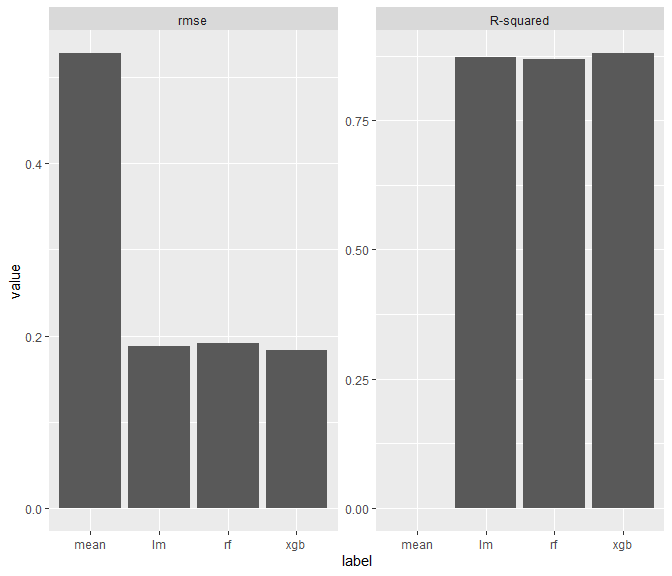plot(perf, fill = "darkred") + xlab(element_blank())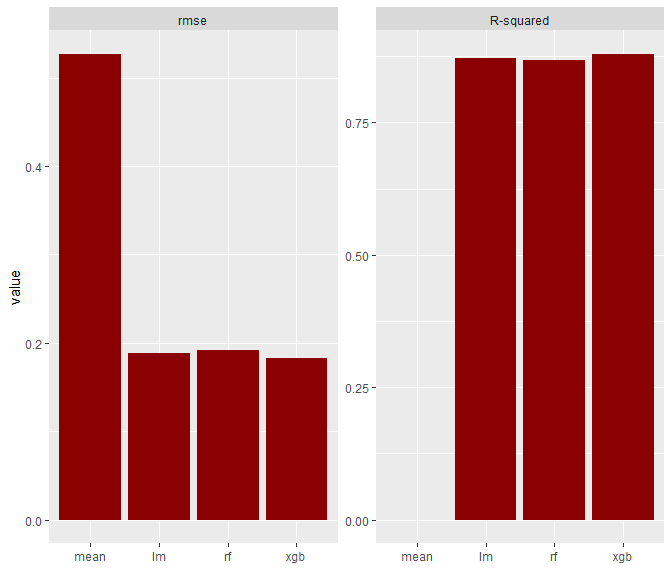The plot “politics” of flashlight is to provide simple graphics with minimal ggplot-tuning, so you are able to add your own modifications. If you are completely unhappy about the proposed plot (e.g. rather favour a scatterplot over a barplot), extract the data slot of perf and create the figure from scratch: head(perf$data)
#> # A tibble: 6 x 3
#>   label metric        value
#>   <fct> <fct>         <dbl>
#> 1 mean  rmse       0.527
#> 2 mean  R-squared -0.000267
#> 3 lm    rmse       0.188
#> 4 lm    R-squared  0.872
#> 5 rf    rmse       0.192
#> 6 rf    R-squared  0.868

perf$data %>% ggplot(aes(x = label, y = value, group = metric, color = metric)) + geom_point()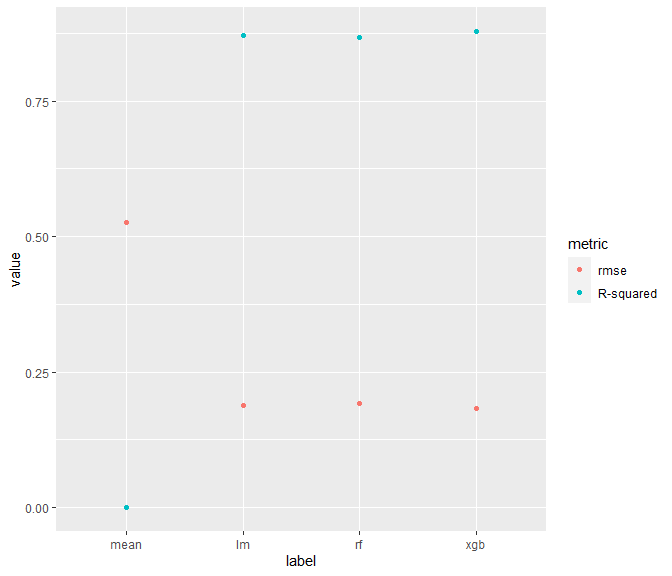The same logic holds for all other main functions in the flashlight package. For performance considerations, the minimum required info in the (multi-)flashlight are: “y”, “predict_function”, “model”, “data” and “metrics”. The latter two can also be passed on the fly. ### Variable importance Now let’s study variable importance of the explainers. (imp <- light_importance(fls, v = x)) #> #> I am an object with class(es) light_importance_multi, light_importance, light, list #> #> data.frames (maximum 6 rows shown): #> #> data #> # A tibble: 6 x 5 #> label metric variable value error #> <fct> <fct> <chr> <dbl> <lgl> #> 1 mean rmse grade 0 NA #> 2 mean rmse year 0 NA #> 3 mean rmse age 0 NA #> 4 mean rmse sqft_living 0 NA #> 5 mean rmse sqft_lot 0 NA #> 6 mean rmse zipcode 0 NA plot(imp, fill = "darkred")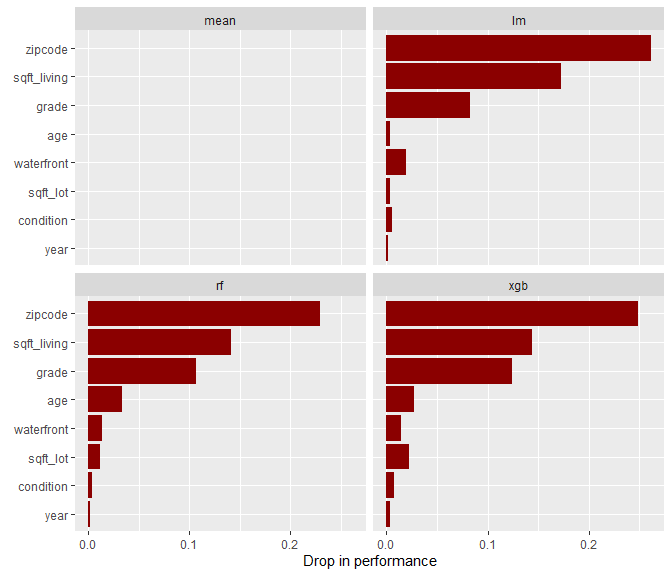Note for permutation importance: If the calculation takes too long (e.g. large query data), set n_max to some reasonable value. light_importance will then randomly pick n_max rows and use only these for assessment of importance. On the other hand, if the calculations is very fast, consider setting m_repetitions to a value > 1 in order to repeat the permutations multiple times. The resulting variable importance corresponds to the average drop in performance and standard errors are added. If SHAP values have been precomputed by add_shap, use type = "shap" to show mean absolute shap values per covariable. ### Individual conditional expectation How do predictions change when sqft_living changes alone? We can investigate this question by looking at “Individual Conditional Expectation” (ICE) profiles of a couple of observations. cp <- light_ice(fls, v = "sqft_living", n_max = 30, seed = 35) plot(cp, alpha = 0.2)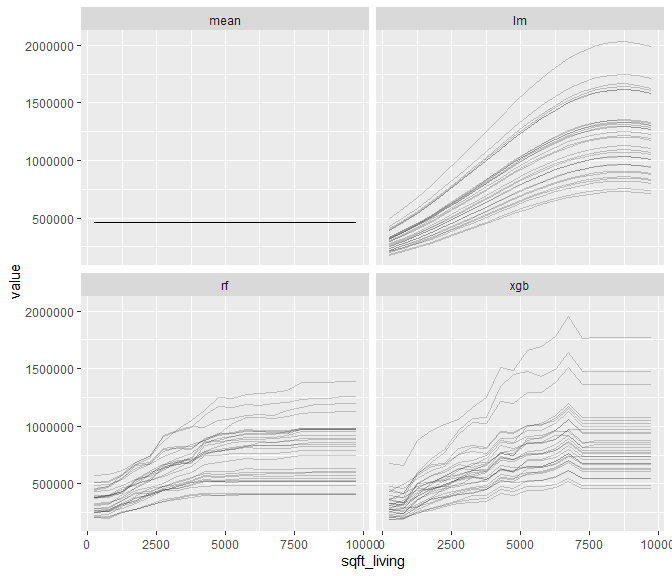Note: Setting seed to a fixed value will ensure that the flashlights will consider the same rows. An alternative would be to pass a small subset of the data to light_ice and calculate all profiles or by passing row indices through indices for fixed selection. Centered ICE profiles (“c-ICE”) can help to increase visibility of interactions. cp <- light_ice(fls, v = "sqft_living", n_max = 30, seed = 35, center = "first") plot(cp, alpha = 0.2)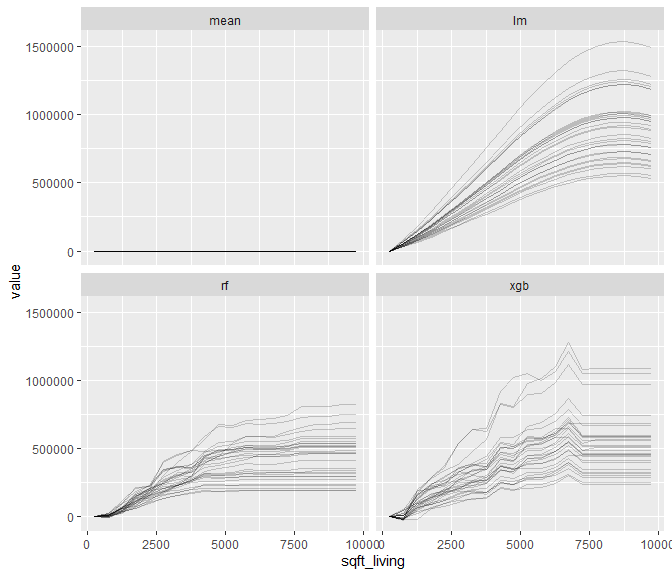### Partial dependence profiles If many ICE profiles (in our case 1000) are averaged, we get an impression on the average effect of the considered variable. Such curves are called partial dependence profiles (PD) resp. partial dependence plots. pd <- light_profile(fls, v = "sqft_living") pd #> #> I am an object with class(es) light_profile_multi, light_profile, light, list #> #> data.frames (maximum 6 rows shown): #> #> data #> # A tibble: 6 x 5 #> sqft_living counts value label type #> <dbl> <int> <dbl> <fct> <fct> #> 1 500 1000 462845. mean partial dependence #> 2 1500 1000 462845. mean partial dependence #> 3 2500 1000 462845. mean partial dependence #> 4 3500 1000 462845. mean partial dependence #> 5 4500 1000 462845. mean partial dependence #> 6 5500 1000 462845. mean partial dependence plot(pd)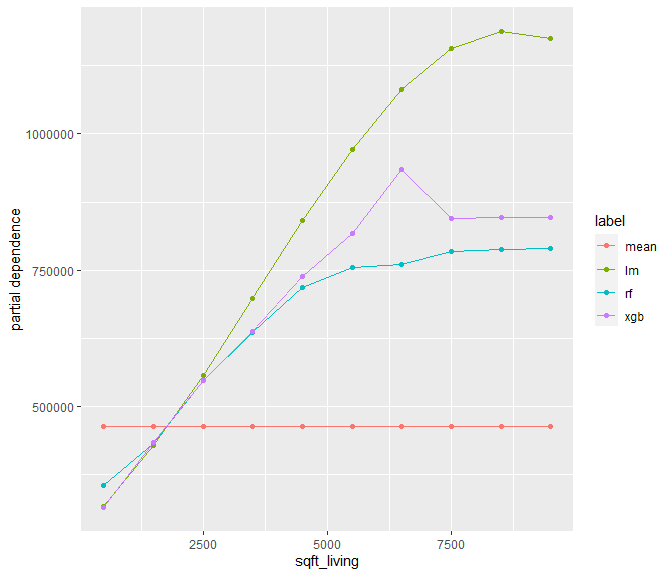The light_profile function offers different ways to specify the evaluation points of the profiles. One option is to explicitly pass such points. pd <- light_profile(fls, v = "sqft_living", pd_evaluate_at = seq(1000, 4000, by = 100)) plot(pd)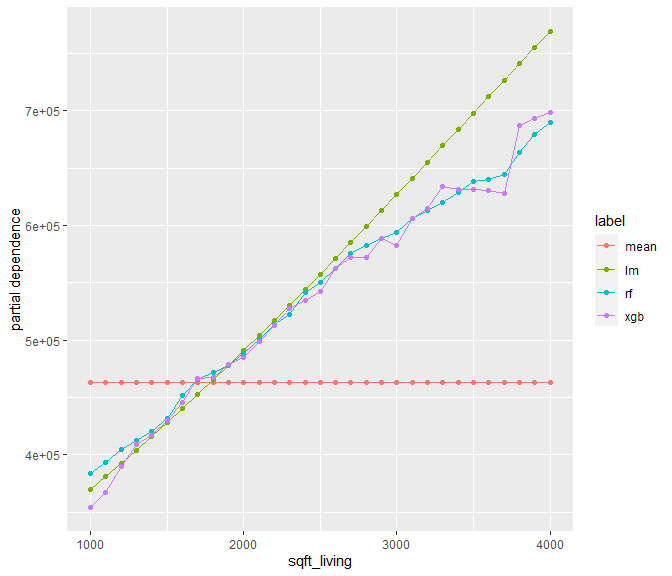For discrete variables: pd <- light_profile(fls, v = "condition") plot(pd)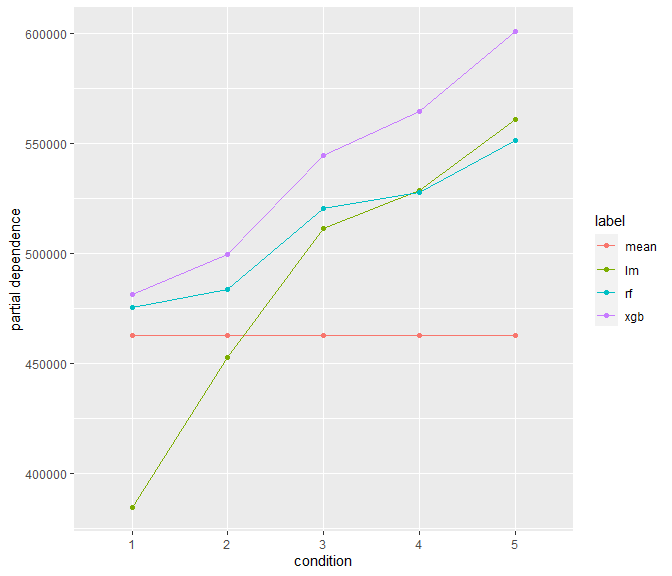### Accumulated local effects (ALE) An approximation of main effects without Ceteris Paribus clause are accumulated local effects profiles (Fisher, Rudin, and Dominici 2018). They are based on accumulating local partial dependence slopes. ale <- light_profile(fls, v = "sqft_living", type = "ale") ale #> #> I am an object with class(es) light_profile_multi, light_profile, light, list #> #> data.frames (maximum 6 rows shown): #> #> data #> # A tibble: 6 x 5 #> sqft_living counts value label type #> <dbl> <int> <dbl> <fct> <fct> #> 1 1500 1000 462845. mean ale #> 2 2500 1000 462845. mean ale #> 3 3500 859 462845. mean ale #> 4 4500 231 462845. mean ale #> 5 5500 52 462845. mean ale #> 6 6500 16 462845. mean ale plot(ale)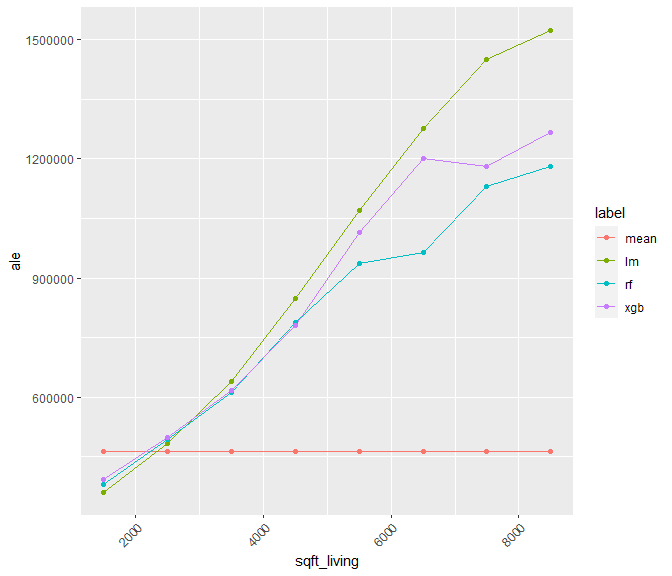Note: While equally sized x-breaks are easy to read, quantile binning usually leads to more stable results. plot(light_profile(fls, v = "sqft_living", type = "ale", cut_type = "quantile"))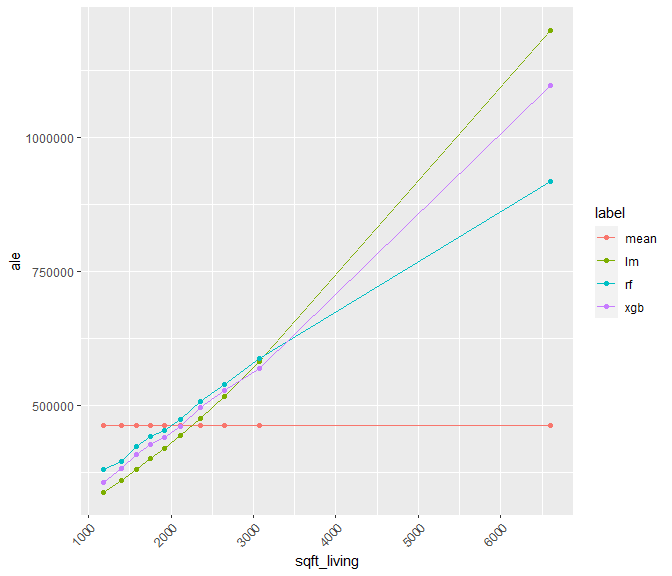In order to calculate ICEs, PDs and ALEs, the following elements need to be available in the (multi-)flashlight: “predict_function”, “model”, “linkinv” and “data”. “data” can also be passed on the fly. ### Profiles of predicted values, residuals, and response We can use the function light_profile not only to create partial dependence profiles but also to get profiles of predicted values (“M plots”), responses or residuals. Additionally, we can either use averages or quartiles as summary statistics. Average predicted values versus the living area are as follows: format_y <- function(x) format(x, big.mark = "'", scientific = FALSE) pvp <- light_profile(fls, v = "sqft_living", type = "predicted", format = "fg", big.mark = "'") plot(pvp) + scale_y_continuous(labels = format_y)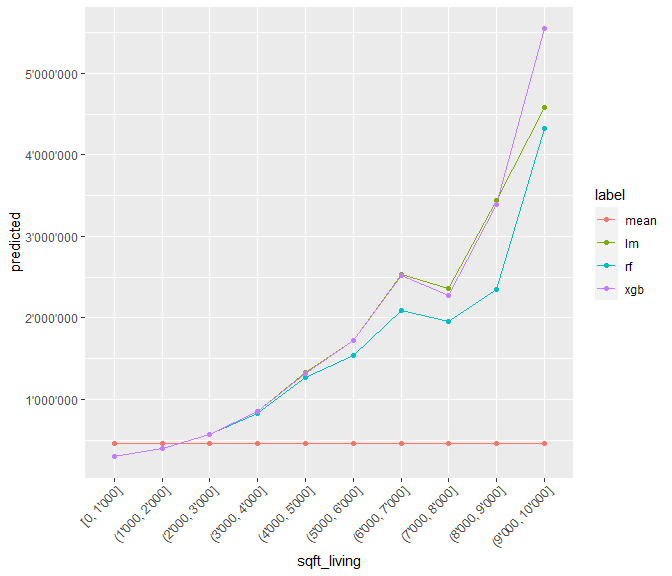Note the formatting of y values as well as the formatC option format = "fg" and big.mark passed to the constructor of the x labels in order to improve basic appearance. We will recycle some of these settings for the next plots. Similarly, the average response profiles (identical for all flashlights, to we only show one of them): rvp <- light_profile(fl_lm, v = "sqft_living", type = "response", format = "fg") plot(rvp) + scale_y_continuous(labels = format_y)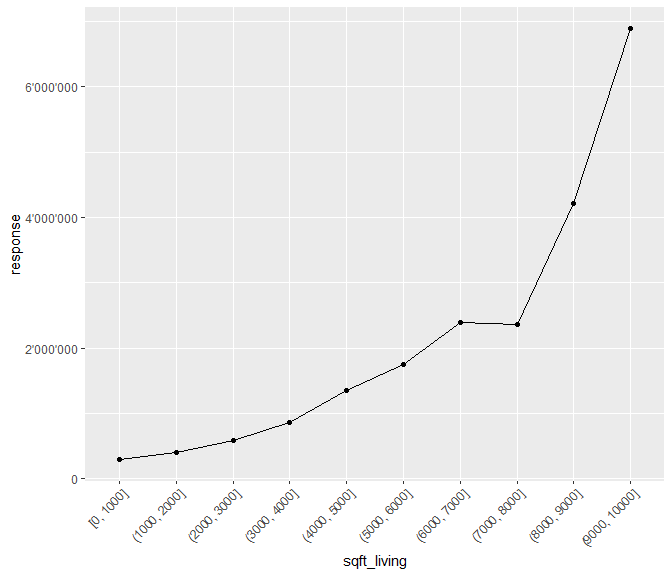Same, but quartiles: rvp <- light_profile(fl_lm, v = "sqft_living", type = "response", stats = "quartiles", format = "fg") plot(rvp) + scale_y_continuous(labels = format_y)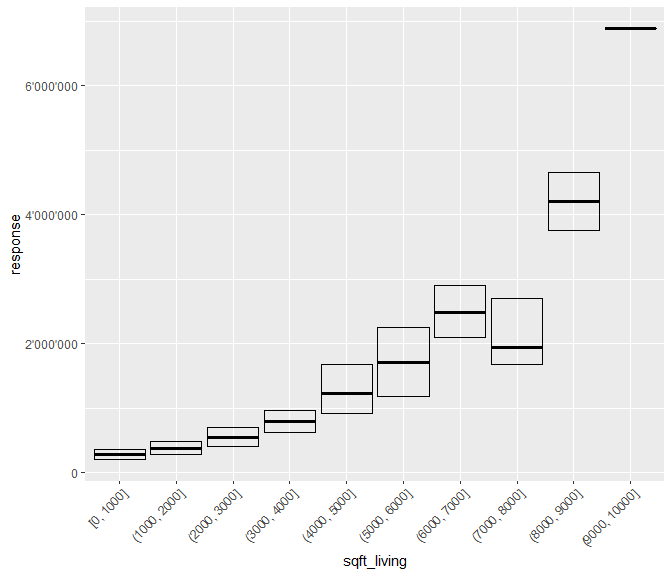What about residuals? First, we remove the “mean” flashlight by setting it NULL. fls$mean <- NULL
rvp <- light_profile(fls, v = "sqft_living", type = "residual",
stats = "quartiles", format = "fg")
plot(rvp) +
scale_y_continuous(labels = format_y)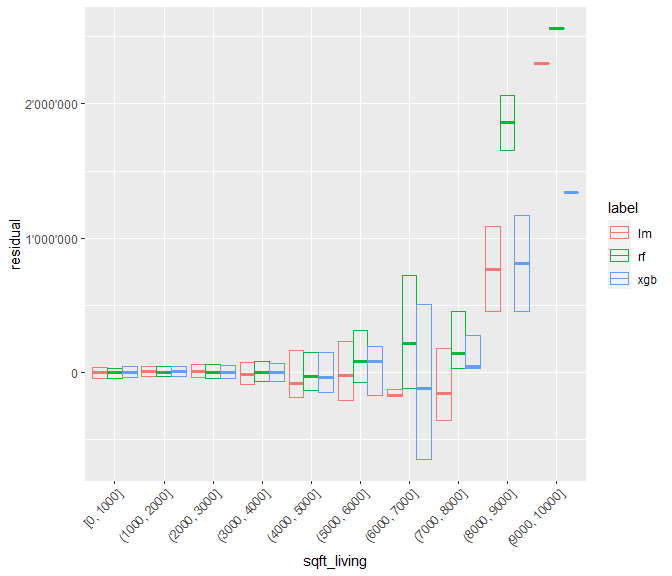While the tree-based models have smaller residuals and medians close to 0, the linear model shows residual curvature that could be captured by representing sqft_living by more parameters.

If your are unhappy about the “group by” strategy, set swap_dim to TRUE.

plot(rvp, swap_dim = TRUE) +
scale_y_continuous(labels = format_y)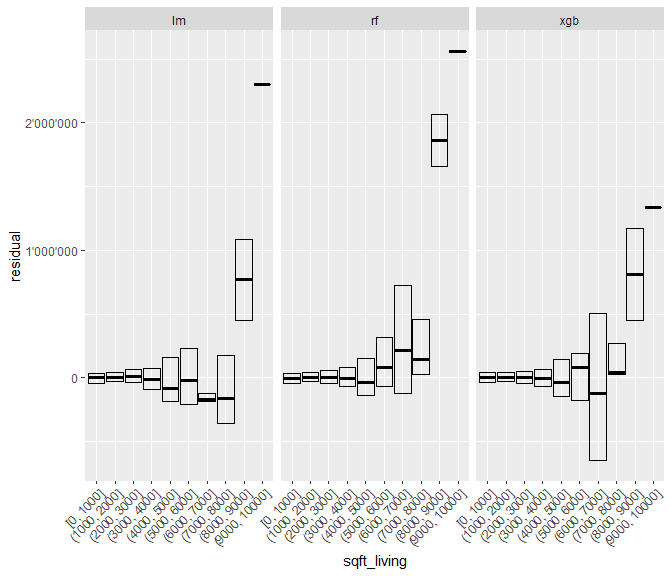For less bars, set n_bins in light_profile:

rvp <- light_profile(fls, v = "sqft_living", type = "residual",
stats = "quartiles", format = "fg", n_bins = 5)
plot(rvp, swap_dim = TRUE) +
scale_y_continuous(labels = format_y)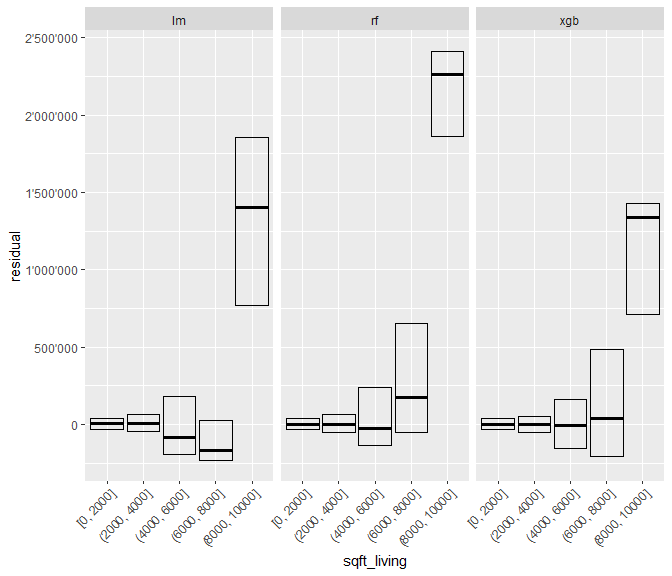Just as diverging ICE profiles give you a clou about the presence of interactions, we can use the option stats = "quartiles" (with pd_center) to show divergence of the mean-centered ICE profiles as boxes (predictions at log-scale to suppress interaction-like effects of the retransformation function):

rvp <- light_profile(fls, v = "sqft_living", use_linkinv = FALSE,
stats = "quartiles", pd_center = "mean")
plot(rvp)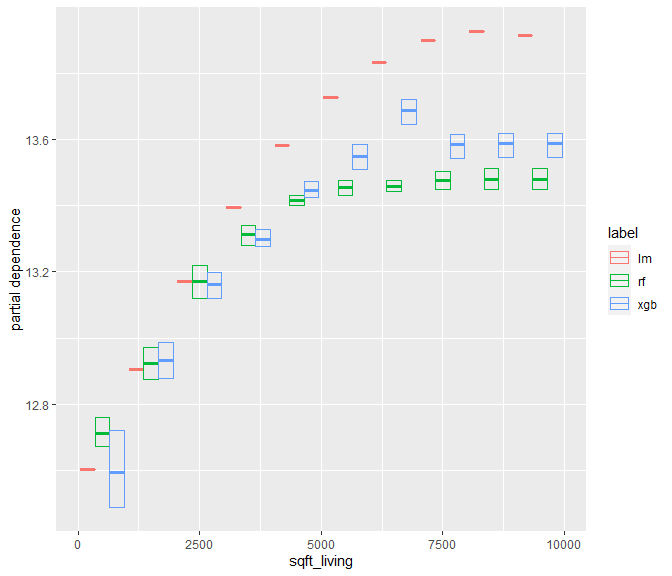For prediction profiles, the same elements as for ICE/PDs are required, while for response profiles we need “y”, “linkinv” and “data”. “data” can also be passed on the fly.

### Visualizing different types of profiles as “effects” plot

In assessing the model quality, it is often useful to visualize

• response profile (quartiles or means),

• average predictions, and

• model effects (partial dependence profiles, or accumulated local effects)

in the same plot and for each input variable. The flashlight package offers the function light_effects to combine such profile plots:

eff <- light_effects(fl_lm, v = "condition")
p <- plot(eff) +
scale_y_continuous(labels = format_y)
p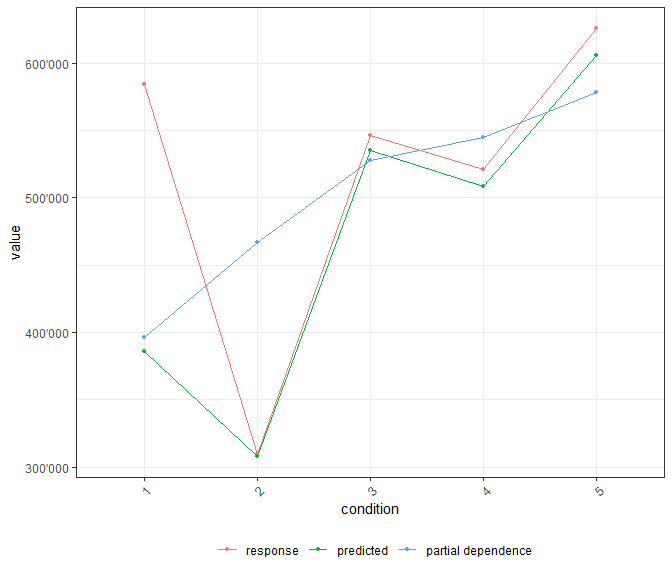Let’s add counts to see if the gaps between response and predicted profiles are problematic or just due to small samples.

plot_counts(p, eff, alpha = 0.2)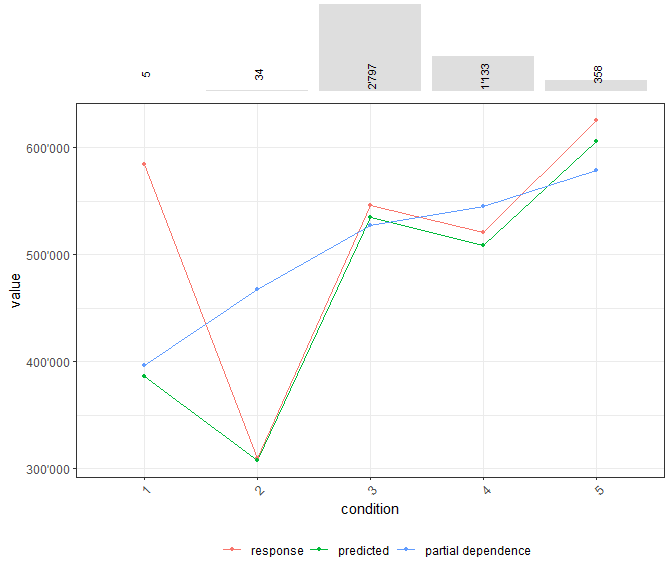The biggest gaps occur at very rare conditions, so the model looks quite fine.

Note: Due to retransformation from log scale, the response profile is slightly higher than the profile of predicted values. If we would evaluate on the modelled log scale, that gap would vanish.

eff <- light_effects(fl_lm, v = "condition", linkinv = I)
p <- plot(eff, use = "all") +
scale_y_continuous(labels = format_y) +
ggtitle("Effects plot on modelled log scale")
p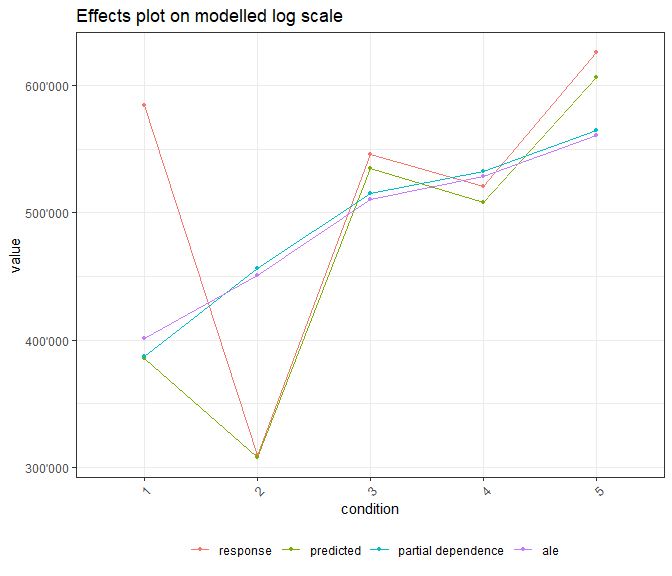Besides adding counts to the figure, representing observed responses as boxplots (no whiskers and outliers in order to avoid too large y scale) might help to judge if there is a problematic misfit.

eff <- light_effects(fl_lm, v = "condition", stats = "quartiles")
p <- plot(eff, rotate_x = FALSE) +
scale_y_continuous(labels = format_y)
plot_counts(p, eff, fill = "blue", alpha = 0.2, width = 0.3)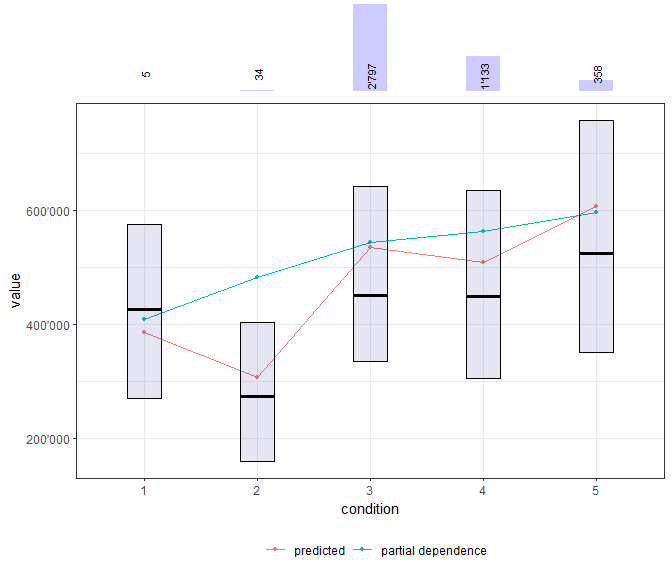The plot method of light_effects allows to hide certain plot elements if it looks too dense. If we would e.g. want to compare partial dependence and accumulated local effects plots, we can use the following approach.

eff <- light_effects(fls, v = "sqft_living", v_labels = FALSE,
cut_type = "quantile", n_bins = 25)
plot(eff, use = c("pd", "ale"), show_points = FALSE) +
scale_y_continuous(labels = format_y) +
coord_cartesian(xlim = c(1000, 4000), ylim = c(3e5, 9e5))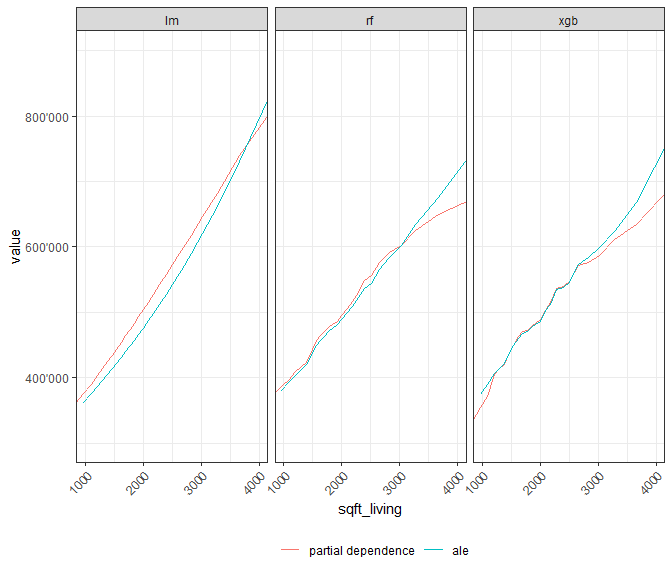As an alternative to profile plots, we could also consider scatter plots.

pr <- light_scatter(fls, v = "sqft_living", n_max = 300)
plot(pr, alpha = 0.2)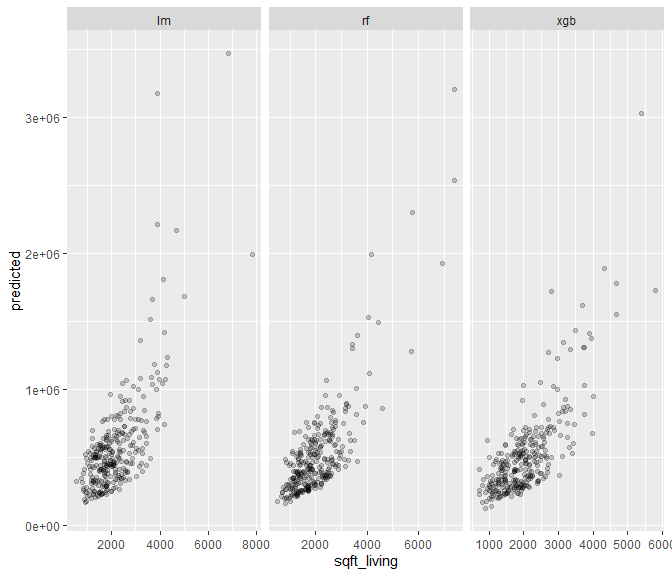### Interaction strength

Based on Friedman’s H statistic (Friedman and Popescu 2008), we can investigate which variable has strongest interaction effect relative to its full effect.

st <- light_interaction(fls, v = x, grid_size = 30, n_max = 50, seed = 42)
plot(st)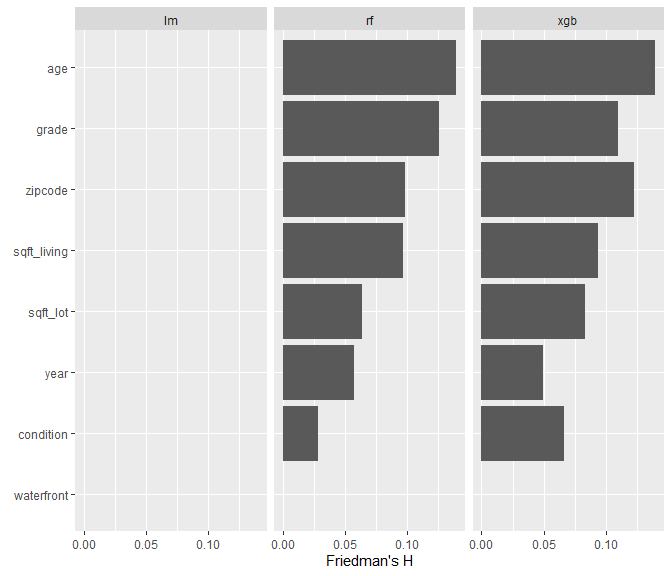Since we have not used interaction terms in the linear model, all values are zero there.

Pairwise interactions are expensive to compute as each variable pair has to be assessed. We limit the search for interactions to the four variables with highest relative interaction strength.

st_pair <- light_interaction(fls, v = most_important(st, 4),
pairwise = TRUE, n_max = 50, seed = 42)
plot(st_pair)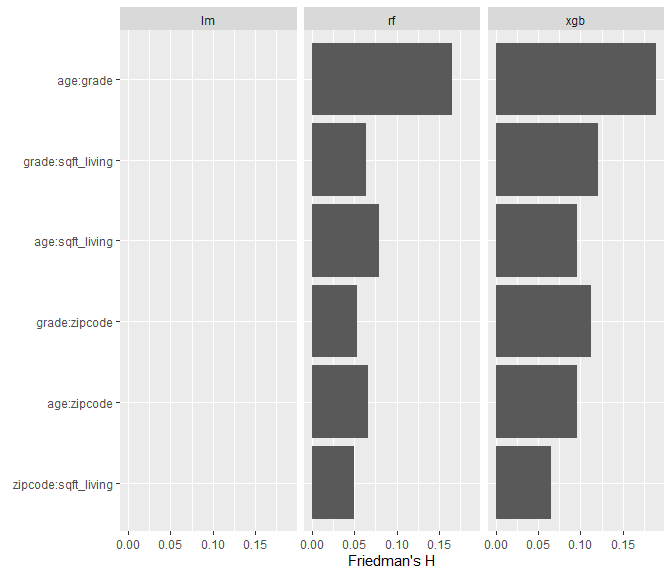### Variable contribution breakdown for single observation

Besides investigating global effects, we can use light_breakdown to calculate variable impact on one single (log) prediction.

bd <- light_breakdown(fl_lm, new_obs = valid[1, ], v = x, n_max = 1000, seed = 74)
plot(bd, size = 3)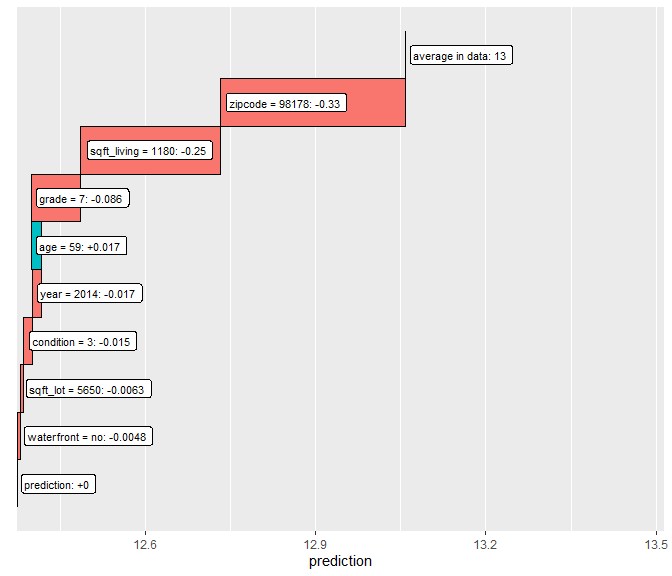We have set the size of the reference data set to n_max = 1000 in order to save time. The only variable with positive impact on the prediction is “age”. The other variables have a negative impact compared to the 1000 reference observations.

### Global surrogate trees

A very different, but intuitive approach is to fit the original predictions of the models by simple decision trees and then visualize these trees.

surr <- light_global_surrogate(fls$xgb, v = x) print(surr$data$r_squared) #>  0.7001644 plot(surr)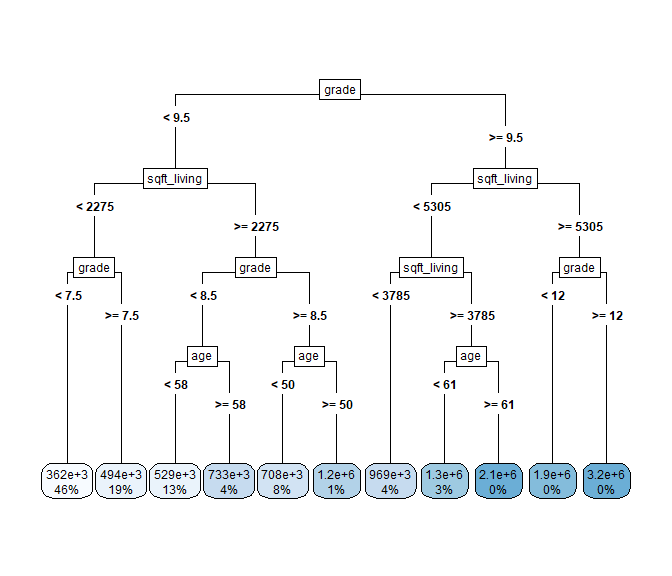Even if it is extremely simple to explain, the surrogate model is not a bad approximation (see R-squared). ## Grouped calculations A key feature of the flashlight package is to support grouped results. You can initialize the (multi-)flashlight with column names of one or many grouping variables or ask for grouped calculations in all major flashlight functions. Plots are adapted accordingly. fls <- multiflashlight(fls, by = "year") # Performance plot(light_performance(fls))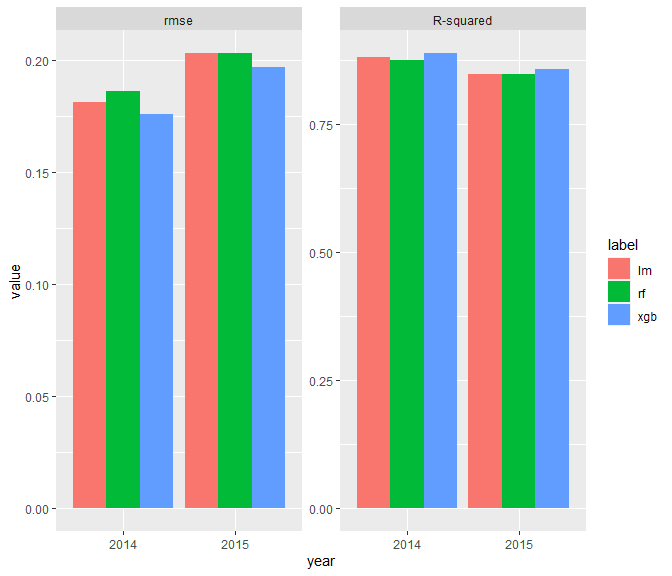# With swapped dimension plot(light_performance(fls), swap_dim = TRUE)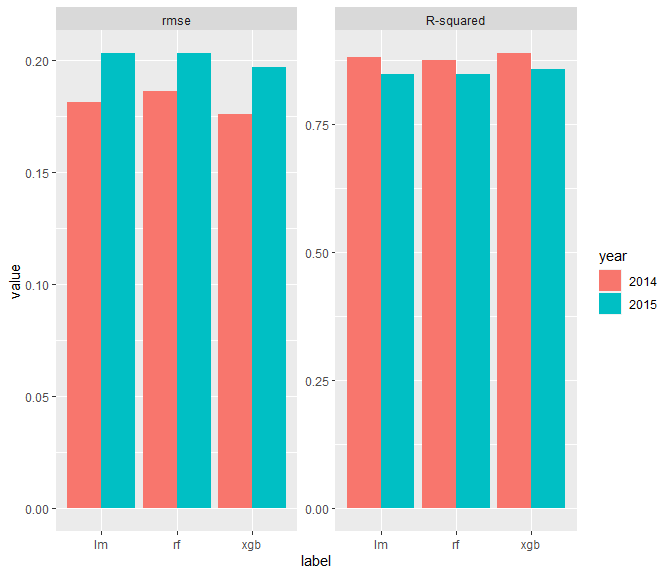# Importance imp <- light_importance(fls, v = x) plot(imp, top_m = 4)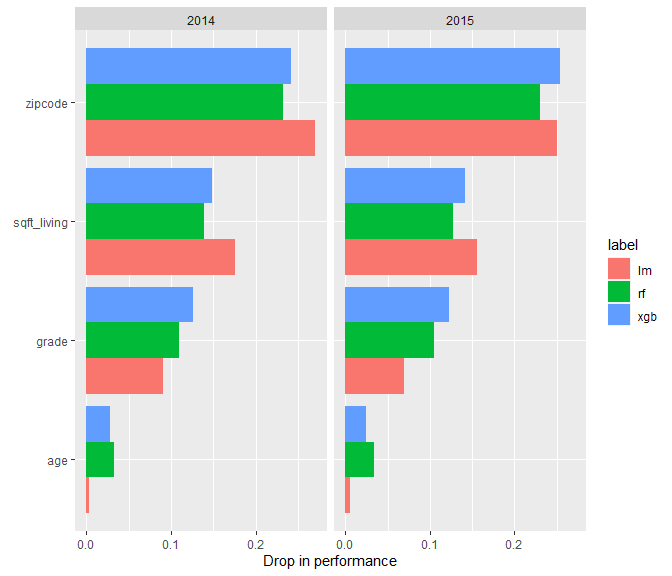plot(imp, swap_dim = TRUE)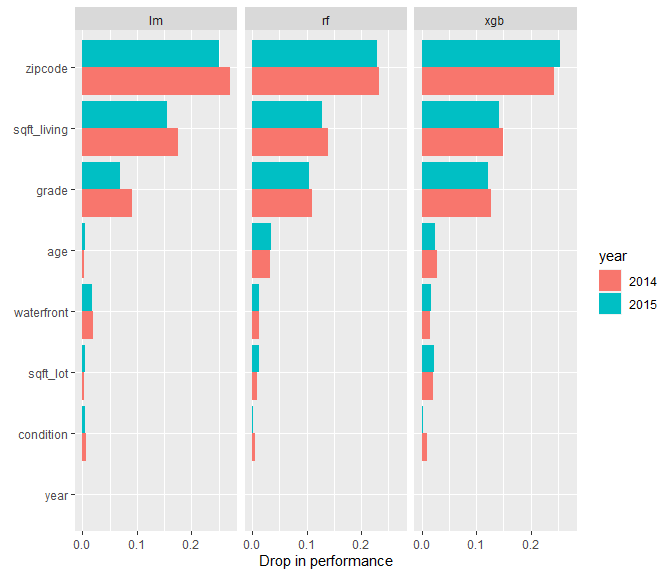# Effects: ICE plot(light_ice(fls, v = "sqft_living", seed = 4345), alpha = 0.8, facet_scales = "free_y") + scale_y_continuous(labels = format_y)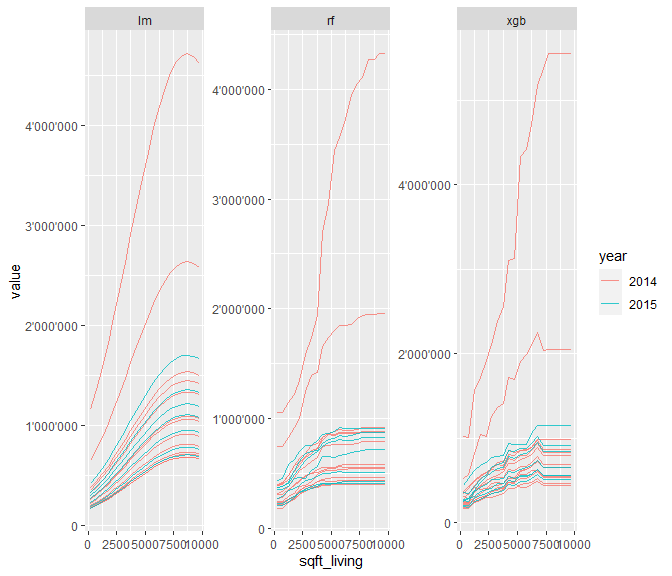# c-ICE plot(light_ice(fls, v = "sqft_living", seed = 4345, center = "middle"), alpha = 0.8, facet_scales = "free_y") + scale_y_continuous(labels = format_y)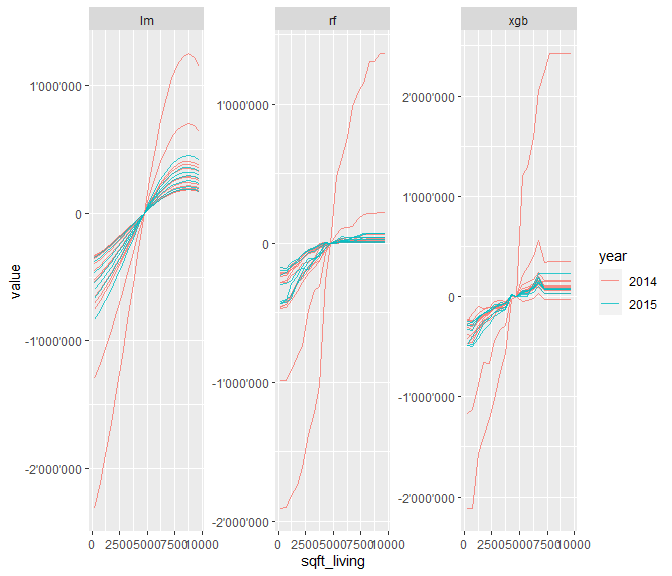# Effects: Partial dependence plot(light_profile(fls, v = "sqft_living"))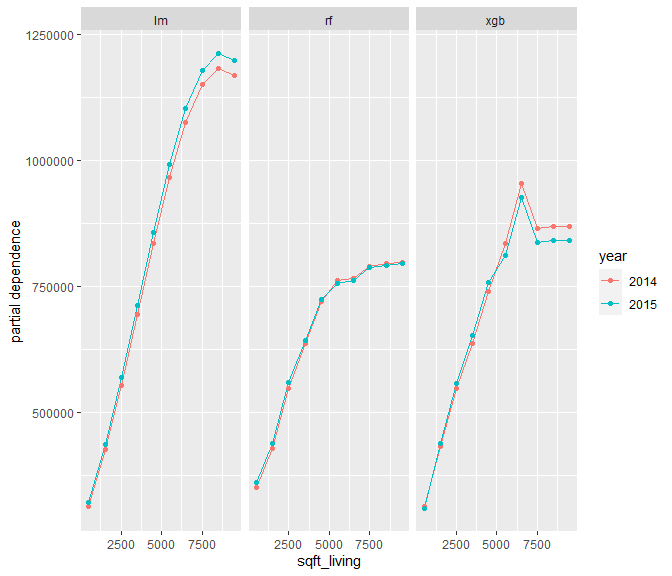plot(light_profile(fls, v = "sqft_living"), swap_dim = TRUE)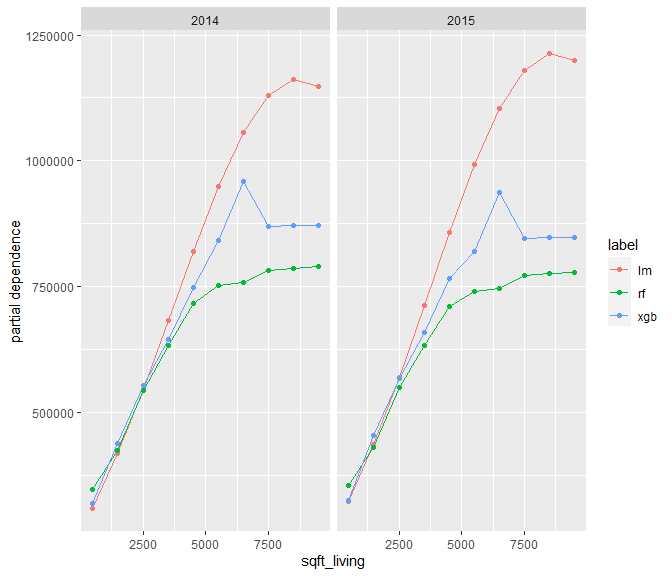plot(light_profile(fls, v = "sqft_living", stats = "quartiles", center = "mean"))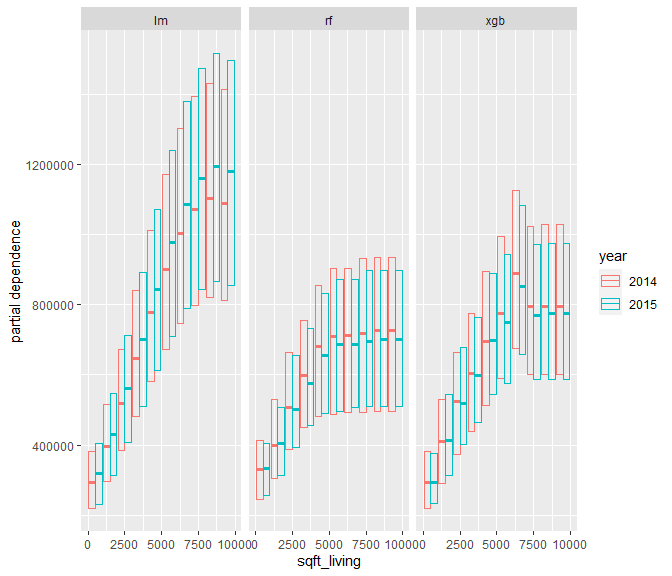# Effects: ALE plot(light_profile(fls, v = "sqft_living", type = "ale", cut_type = "quantile"))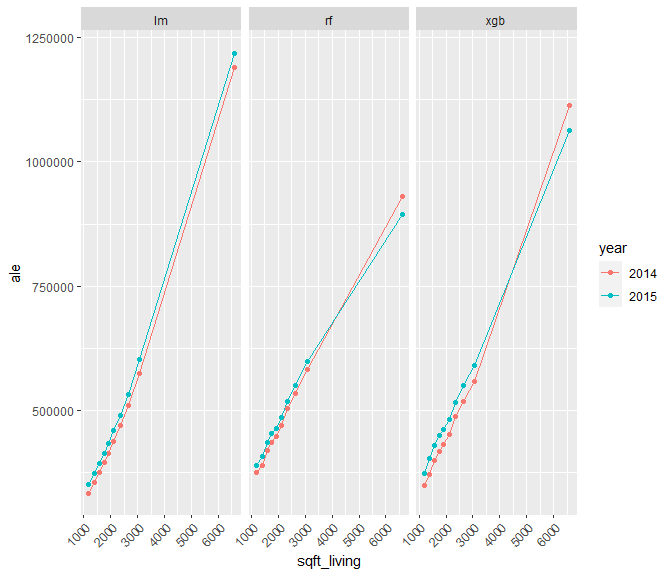plot(light_profile(fls, v = "sqft_living", type = "ale", cut_type = "quantile"), swap_dim = TRUE)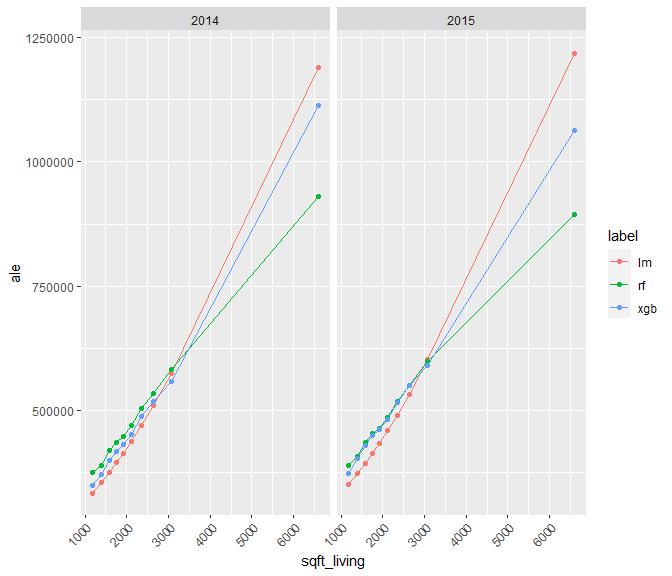# Effects: Combined plot (only one flashlight) # -> we need to manually pass "by" or update the single flashlight z <- light_effects(fls, v = "sqft_living", format = "fg", stats = "quartiles", n_bins = 5, by = NULL) p <- plot(z) + scale_y_continuous(labels = format_y) + coord_cartesian(ylim = c(0, 3e6)) plot_counts(p, z, alpha = 0.2)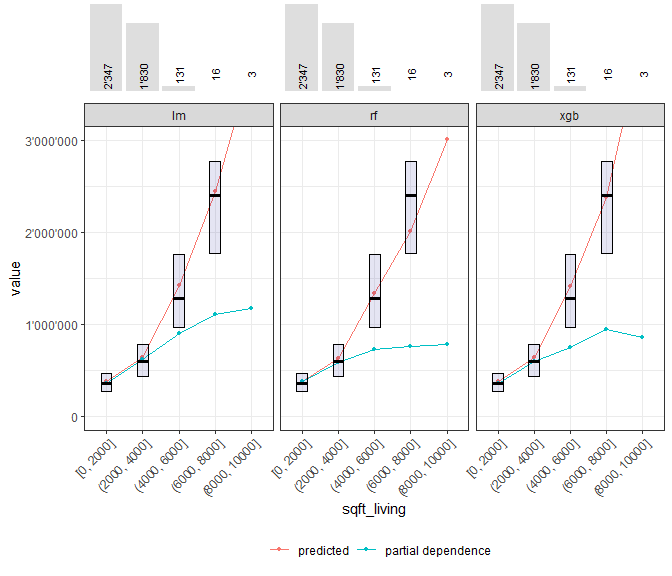# Scatter pr <- light_scatter(fls, v = "sqft_living", n_max = 300) plot(pr, alpha = 0.8)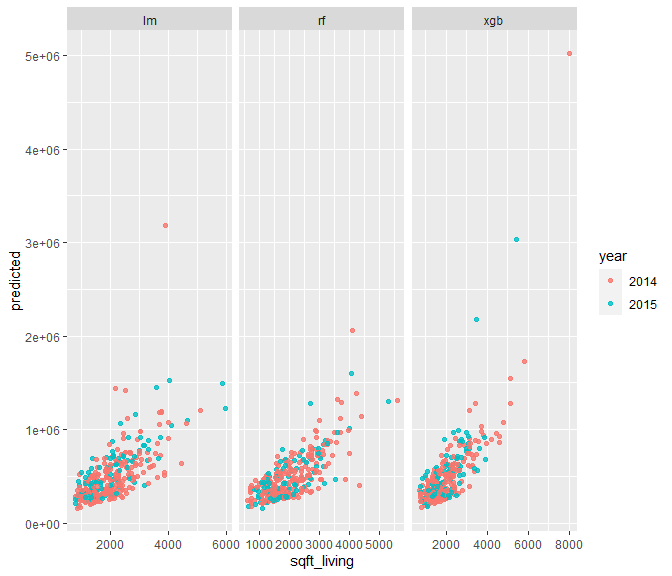# Variable contribution breakdown for single observation (on log-scale) # -> "by" selects the relevant rows in data/valid plot(light_breakdown(fl_lm, new_obs = valid[1, ], v = x, top_m = 3))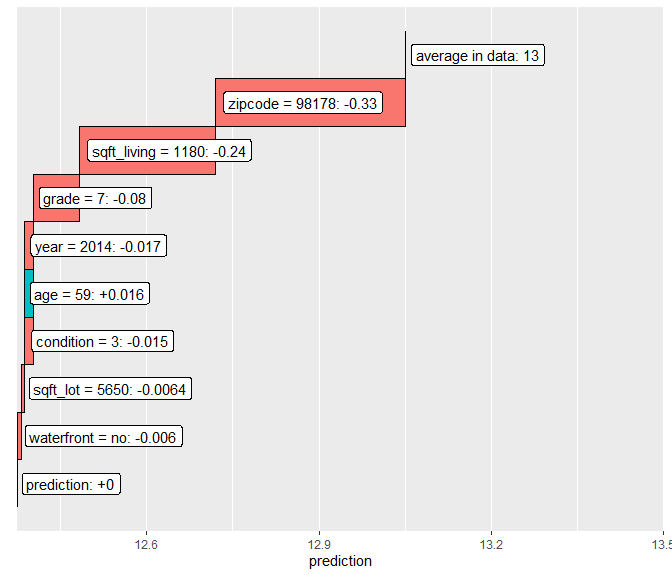# Global surrogate plot(light_global_surrogate(fls$xgb, v = x, maxdepth = 3))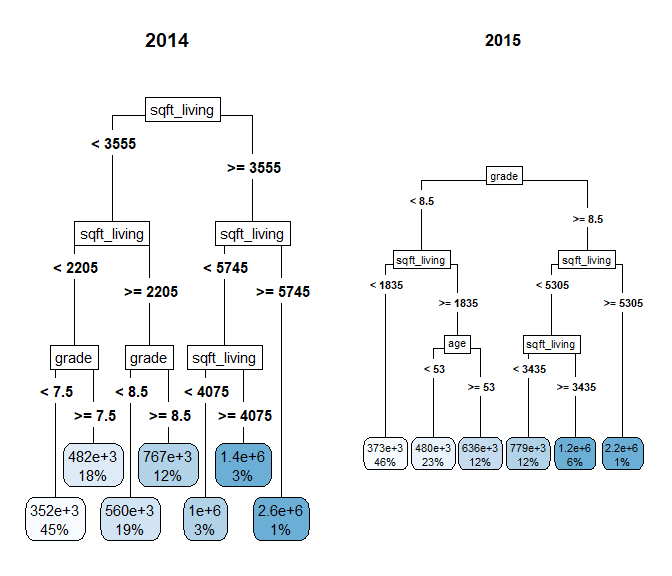## Working with case weights

In many applications, case weights need to be taken into account. All main functions in flashlight are able to deal with them. The only thing you need to do is to pass the column name of the case weights to w when initializing the (multi-)flashlight.

Let’s go through the initial iris example again with (artificial) case weights:

# Add weight info to the flashlight
fl_weighted <- flashlight(fl, w = "Petal.Length", label = "ols weighted")
fls <- multiflashlight(list(fl, fl_weighted))

# Performance: rmse and R-squared
plot(light_performance(fls))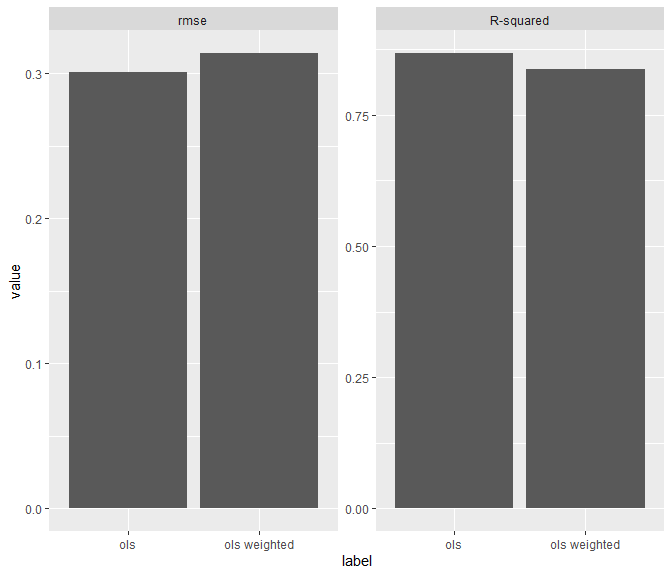plot(light_performance(fls, by = "Species"))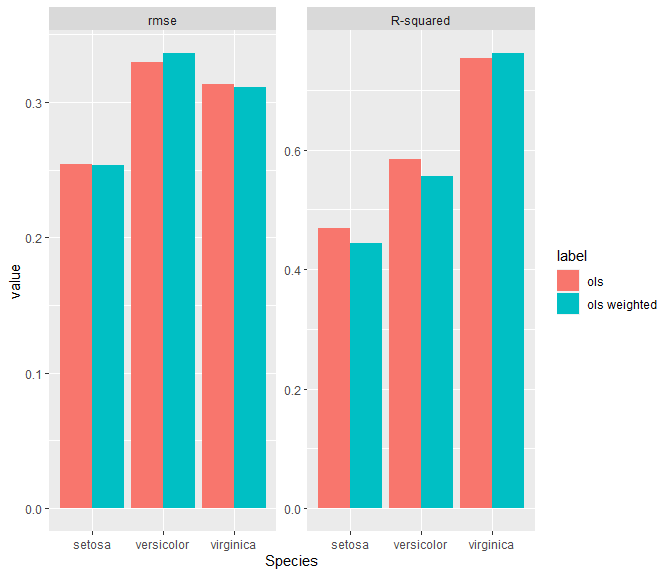# Variable importance by drop in rmse
plot(light_importance(fls, by = "Species"))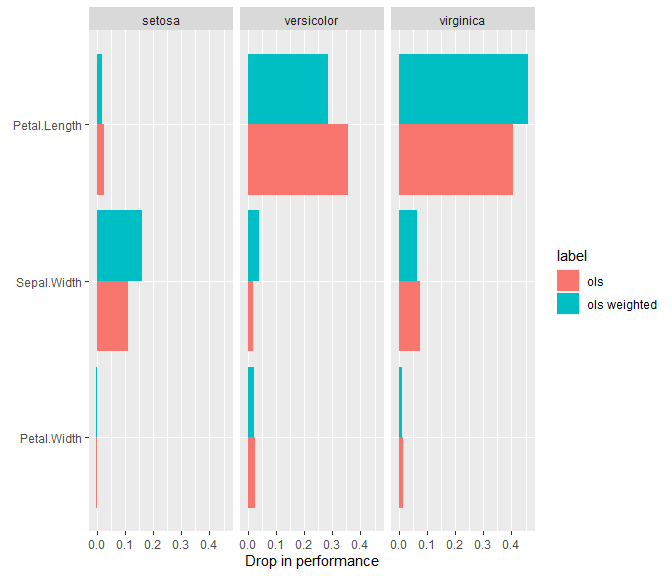# ICE profiles for Petal.Width
# (not affected by weights because nothing is being aggregated)
indices <- seq(10, 150, by = 10)
plot(light_ice(fls, v = "Petal.Width", indices = indices), alpha = 0.2)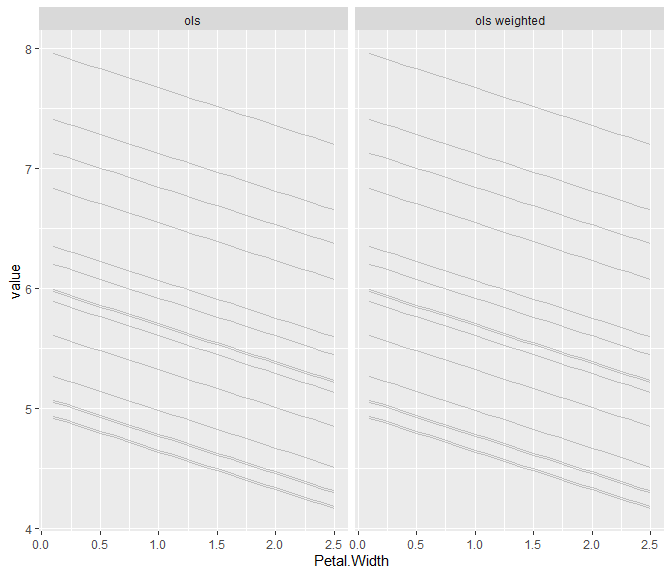plot(light_ice(fls, v = "Petal.Width", by = "Species", indices = indices))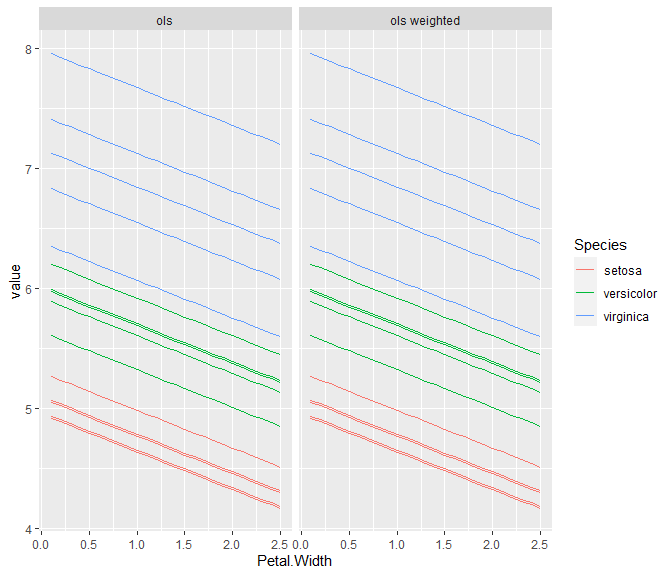# c-ICE -> lines overlap, no interactions at all
plot(light_ice(fls, v = "Petal.Width", indices = indices, center = "first"), alpha = 0.2)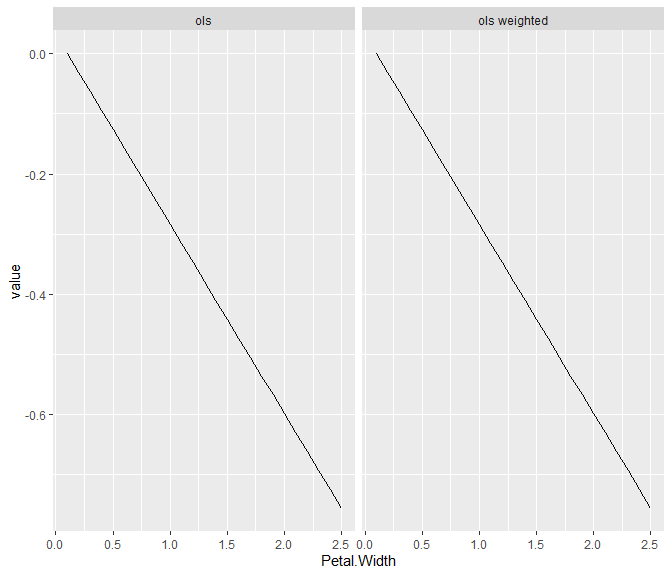plot(light_ice(fls, v = "Petal.Width", by = "Species", indices = indices, center = "first"))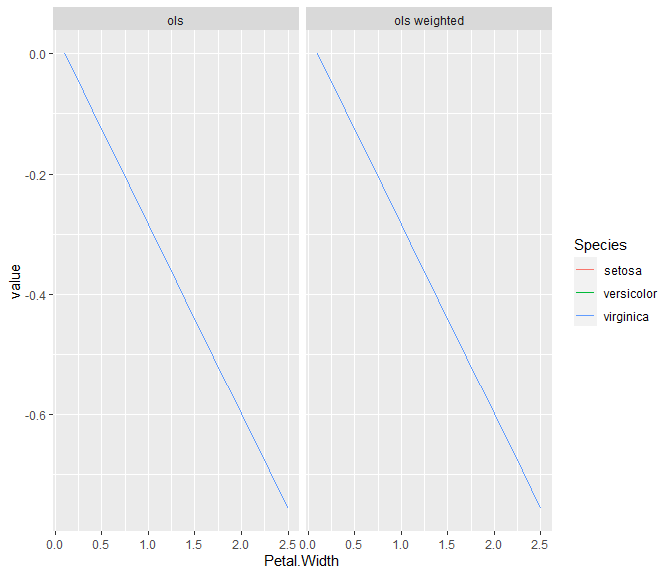# Partial dependence profiles for Petal.Width
plot(light_profile(fls, v = "Petal.Width"))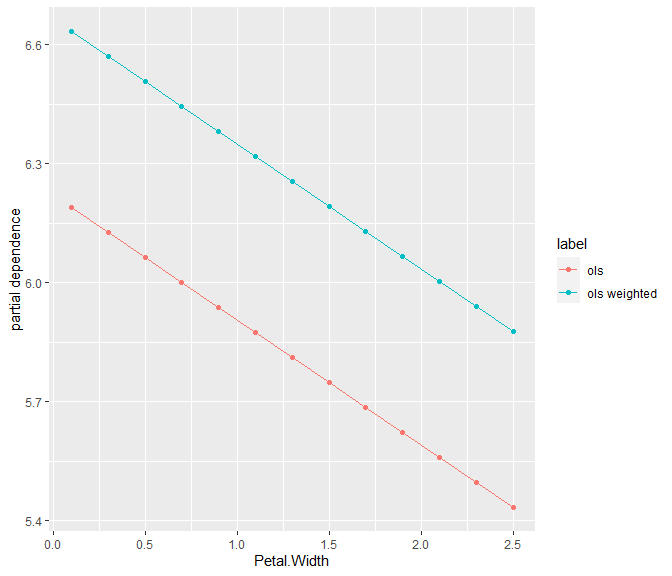plot(light_profile(fls, v = "Petal.Width", by = "Species"))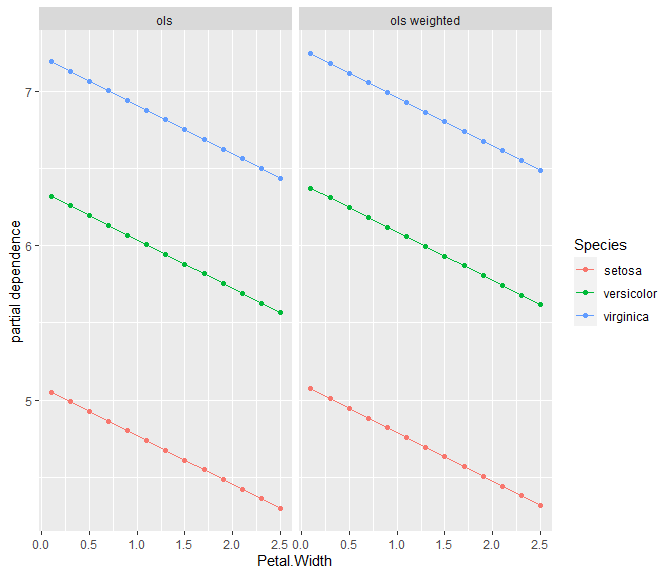# ALE profiles for Petal.Width
plot(light_profile(fls, v = "Petal.Width", type = "ale"))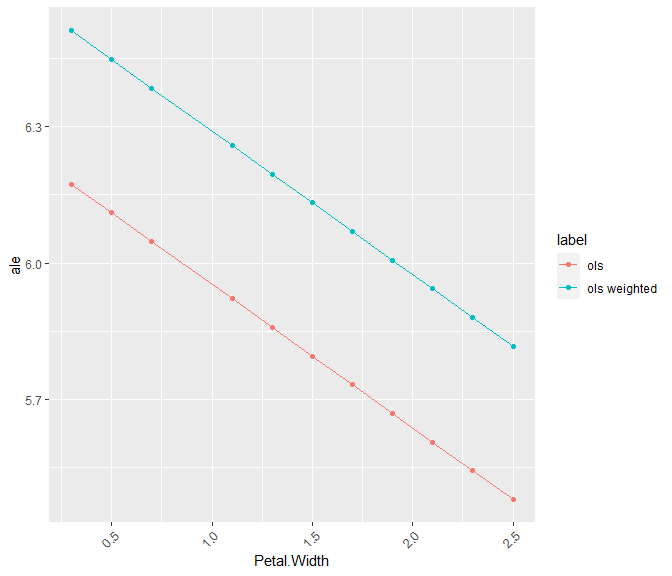plot(light_profile(fls, v = "Petal.Width", by = "Species", type = "ale"))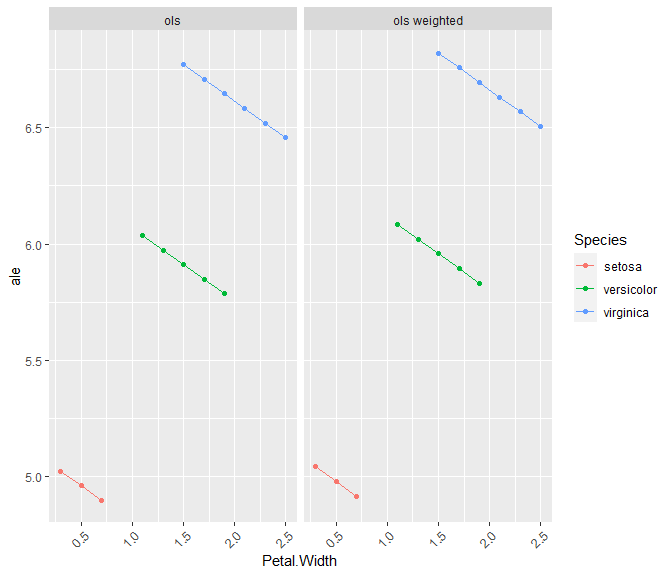# Observed, predicted, and partial dependence profiles
plot(light_effects(fls, v = "Petal.Width"))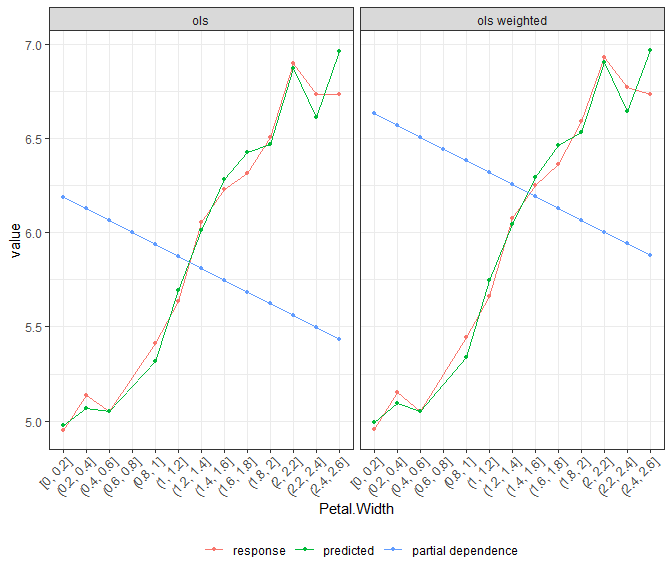eff <- light_effects(fls, v = "Petal.Width", stats = "quartiles")
plot(eff) %>%
plot_counts(eff, alpha = 0.2, fill = "blue")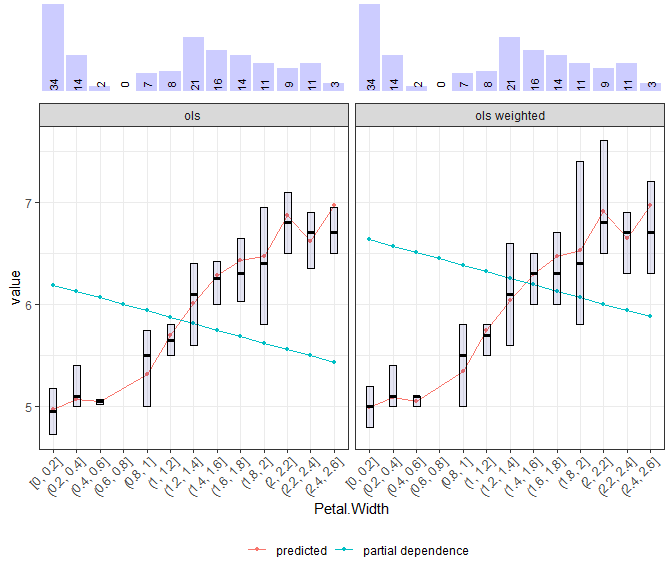# Variable contribution breakdown for single observation (on log-scale)
plot(light_breakdown(fls, new_obs = iris[2, ]), size = 2.5)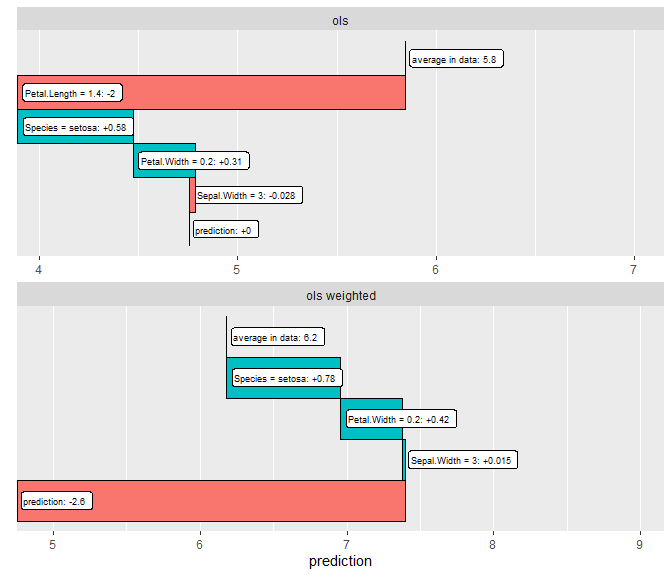# Global surrogate
plot(light_global_surrogate(fls))